We want to identify biodiversity refuge zones in Madagascar

## 2 Datasets

### 2.1 Forest inventory

National forest inventories carried out on $$753$$ sites on the island of Madagascar are available, listing the presence or absence of $$555$$ plant species on each of these sites between 1994 and 1996. We use these forest inventories to calculate a matrix indicating the presence by a $$1$$ and the absence by a $$0$$ of the species at each site by removing observations for which the species is not identified. This matrix therefore records the occurrences of $$483$$ species at $$751$$ sites.

# forest inventory
trees <- trees[-which(is.na(trees$sp)),] trees$plot <- as.character(trees$plot) plots <- unique(trees$plot)
nplot <- length(plots)
sp <- unique(trees$taxize) nsp <- length(sp) # presence/absence of each species on each plot nplot <- length(unique(trees$plot))
species <- unique(trees$taxize) nsp <- length(species) # presence/absence of each species on each plot PA <- matrix(0, nplot, nsp) rownames(PA) <- paste(sort(as.numeric(unique(trees$plot))))
colnames(PA) <- paste0("sp_", 1:nsp)
for (i in 1:nplot){
for (j in 1:nsp){
idx <- which(trees$taxize == species[j]) PA[paste(trees$plot[idx]),j] <- 1
}
}
# save presence/absence at inventory sites matrix
save(madagascar, file="~/Documents/jSDM/data/madagascar.rda")
data(madagascar, package="jSDM")
#>   sp_1 sp_2 sp_3 sp_4 sp_5 sp_6 sp_7 sp_8 sp_9 sp_10 sp_11 sp_12 sp_13 sp_14
#> 1    0    0    0    0    0    0    0    0    0     0     0     0     0     0
#> 2    0    0    0    0    0    1    0    0    0     0     0     0     0     0
#> 3    0    0    0    0    0    0    0    0    1     0     0     0     0     0
#> 4    0    0    0    0    0    0    0    0    0     0     0     0     0     0
#> 5    0    0    0    0    0    0    0    0    0     0     0     0     0     0
#> 6    0    0    0    1    0    0    0    0    0     0     0     0     0     1
#>   sp_15 sp_16 sp_17 sp_18 sp_19 sp_20 sp_21 sp_22 sp_23 sp_24 sp_25 sp_26 sp_27
#> 1     0     0     0     0     0     0     1     0     0     0     0     0     0
#> 2     0     0     0     0     0     0     0     1     0     0     0     0     0
#> 3     0     0     0     0     0     0     0     1     0     0     0     0     0
#> 4     0     0     0     0     0     0     1     0     0     0     0     0     0
#> 5     0     0     0     0     0     0     0     1     0     0     0     0     0
#> 6     0     0     0     0     0     0     1     1     0     0     0     0     0
#>   sp_28 sp_29 sp_30 sp_31 sp_32 sp_33 sp_34 sp_35 sp_36 sp_37 sp_38 sp_39 sp_40
#> 1     0     0     0     0     0     0     0     0     0     0     0     0     0
#> 2     0     0     0     0     0     0     0     0     0     0     0     0     0
#> 3     0     0     0     0     0     0     0     0     0     0     0     0     0
#> 4     0     0     0     0     0     0     0     0     0     0     0     0     0
#> 5     0     0     0     0     0     0     0     0     0     0     0     0     0
#> 6     0     0     0     0     0     0     0     0     0     0     0     0     0
#>   sp_41 sp_42 sp_43 sp_44 sp_45 sp_46 sp_47 sp_48 sp_49 sp_50 sp_51 sp_52 sp_53
#> 1     0     0     0     1     0     0     0     0     0     0     0     0     0
#> 2     0     0     0     0     0     0     0     1     0     0     0     0     0
#> 3     0     0     0     0     0     0     0     0     0     0     0     0     0
#> 4     0     0     0     0     0     0     0     0     0     0     0     0     0
#> 5     0     0     0     0     0     0     0     0     0     0     0     0     0
#> 6     0     1     0     0     0     0     0     0     0     0     0     0     0
#>   sp_54 sp_55 sp_56 sp_57 sp_58 sp_59 sp_60 sp_61 sp_62 sp_63 sp_64 sp_65 sp_66
#> 1     0     0     0     0     0     0     0     0     0     0     0     0     0
#> 2     0     0     0     0     0     0     0     0     0     0     0     0     0
#> 3     0     0     0     0     0     0     0     0     0     0     0     0     0
#> 4     1     0     0     0     0     0     0     0     0     0     0     0     0
#> 5     0     0     0     0     0     0     0     0     0     0     1     0     0
#> 6     0     0     0     0     0     0     0     0     0     0     0     0     0
#>   sp_67 sp_68 sp_69 sp_70 sp_71 sp_72 sp_73 sp_74 sp_75 sp_76 sp_77 sp_78 sp_79
#> 1     0     0     0     0     0     0     0     0     0     0     0     0     0
#> 2     0     0     0     0     0     0     0     0     0     0     0     0     0
#> 3     0     0     0     0     0     0     0     0     0     0     0     0     0
#> 4     0     0     0     0     0     0     0     0     0     0     0     1     0
#> 5     0     0     0     0     0     0     0     0     0     0     0     0     0
#> 6     0     0     0     0     0     0     0     0     0     0     0     0     0
#>   sp_80 sp_81 sp_82 sp_83 sp_84 sp_85 sp_86 sp_87 sp_88 sp_89 sp_90 sp_91 sp_92
#> 1     0     0     0     0     1     0     0     0     0     0     0     1     0
#> 2     0     0     0     0     0     0     0     0     0     0     0     0     0
#> 3     0     0     0     0     0     0     0     0     0     0     0     0     0
#> 4     0     0     0     0     0     0     0     0     0     0     0     0     0
#> 5     0     0     0     0     0     0     0     0     0     0     0     0     0
#> 6     0     0     0     0     0     0     0     0     0     0     0     0     0
#>   sp_93 sp_94 sp_95 sp_96 sp_97 sp_98 sp_99 sp_100 sp_101 sp_102 sp_103 sp_104
#> 1     0     0     0     0     0     0     0      0      0      0      0      0
#> 2     0     0     0     0     0     0     0      0      0      0      0      0
#> 3     0     0     1     0     0     0     0      0      0      0      0      0
#> 4     0     0     0     0     0     0     0      0      0      0      0      0
#> 5     0     0     0     0     0     0     0      0      0      0      0      0
#> 6     0     0     1     0     0     0     0      0      0      0      0      0
#>   sp_105 sp_106 sp_107 sp_108 sp_109 sp_110 sp_111 sp_112 sp_113 sp_114 sp_115
#> 1      0      0      0      0      0      0      0      0      0      0      0
#> 2      0      0      0      0      0      1      0      0      0      0      0
#> 3      0      0      0      0      0      0      0      0      0      0      0
#> 4      0      0      0      0      0      0      0      0      0      0      0
#> 5      0      0      0      0      0      0      0      0      0      0      0
#> 6      0      0      0      0      0      0      0      0      0      0      0
#>   sp_116 sp_117 sp_118 sp_119 sp_120 sp_121 sp_122 sp_123 sp_124 sp_125 sp_126
#> 1      0      0      0      0      0      0      0      0      0      0      0
#> 2      0      0      0      0      0      0      0      0      0      0      0
#> 3      0      0      0      0      0      0      0      0      0      0      0
#> 4      0      0      0      0      0      0      0      0      0      0      0
#> 5      0      0      0      0      0      0      0      0      0      0      0
#> 6      0      0      0      0      0      0      0      0      0      0      0
#>   sp_127 sp_128 sp_129 sp_130 sp_131 sp_132 sp_133 sp_134 sp_135 sp_136 sp_137
#> 1      0      0      0      0      0      0      0      0      0      0      0
#> 2      0      0      0      0      0      0      0      0      0      0      0
#> 3      0      0      0      0      0      0      0      0      0      0      0
#> 4      0      0      0      0      0      0      0      0      0      0      0
#> 5      0      0      0      0      0      0      0      0      0      0      0
#> 6      0      0      1      0      0      0      0      0      0      0      0
#>   sp_138 sp_139 sp_140 sp_141 sp_142 sp_143 sp_144 sp_145 sp_146 sp_147 sp_148
#> 1      0      0      0      0      0      0      0      0      1      0      1
#> 2      0      0      0      0      0      0      0      0      0      1      1
#> 3      0      0      0      0      0      0      0      0      0      0      0
#> 4      0      0      0      0      0      0      0      0      1      1      1
#> 5      0      0      0      0      0      0      0      0      1      0      1
#> 6      0      0      0      0      0      0      0      0      0      0      0
#>   sp_149 sp_150 sp_151 sp_152 sp_153 sp_154 sp_155 sp_156 sp_157 sp_158 sp_159
#> 1      0      0      0      0      0      1      1      1      0      0      0
#> 2      0      0      1      0      0      0      0      0      0      0      1
#> 3      1      1      1      1      1      0      0      0      0      0      0
#> 4      0      0      0      0      0      0      0      0      0      0      0
#> 5      0      1      0      0      0      0      0      0      1      1      0
#> 6      0      0      0      0      0      0      0      0      0      0      0
#>   sp_160 sp_161 sp_162 sp_163 sp_164 sp_165 sp_166 sp_167 sp_168 sp_169 sp_170
#> 1      0      0      0      0      0      0      0      0      0      0      0
#> 2      1      0      0      0      0      0      0      0      0      0      0
#> 3      0      0      0      0      0      0      0      0      0      0      0
#> 4      0      0      0      0      0      0      0      0      0      0      0
#> 5      0      0      0      0      0      0      0      0      0      0      0
#> 6      0      1      0      1      0      0      0      0      0      0      0
#>   sp_171 sp_172 sp_173 sp_174 sp_175 sp_176 sp_177 sp_178 sp_179 sp_180 sp_181
#> 1      0      0      0      0      0      0      0      0      0      0      0
#> 2      0      0      0      0      0      0      0      0      0      0      0
#> 3      0      0      0      0      0      0      0      0      0      0      0
#> 4      0      0      0      0      0      0      0      0      0      0      0
#> 5      0      0      0      0      0      0      0      0      0      0      0
#> 6      0      0      0      0      0      0      0      0      0      0      0
#>   sp_182 sp_183 sp_184 sp_185 sp_186 sp_187 sp_188 sp_189 sp_190 sp_191 sp_192
#> 1      0      0      0      0      0      0      0      0      0      0      0
#> 2      0      0      0      0      0      0      0      0      0      0      0
#> 3      0      0      0      0      0      0      0      0      0      0      0
#> 4      0      0      0      0      0      0      0      0      0      0      0
#> 5      0      0      0      0      0      0      0      0      0      0      0
#> 6      0      0      0      0      0      0      0      0      0      0      0
#>   sp_193 sp_194 sp_195 sp_196 sp_197 sp_198 sp_199 sp_200 sp_201 sp_202 sp_203
#> 1      0      0      0      0      0      0      0      0      0      0      0
#> 2      0      0      0      0      0      0      0      0      0      0      0
#> 3      0      0      0      0      0      0      0      0      0      0      0
#> 4      0      0      0      0      0      0      0      0      0      0      0
#> 5      0      0      0      0      0      0      0      0      0      0      0
#> 6      0      0      0      0      0      0      0      0      0      0      0
#>   sp_204 sp_205 sp_206 sp_207 sp_208 sp_209 sp_210 sp_211 sp_212 sp_213 sp_214
#> 1      0      0      0      0      0      0      0      0      0      0      0
#> 2      0      0      0      0      0      0      0      0      0      0      0
#> 3      0      0      0      0      0      0      0      0      0      0      0
#> 4      0      0      0      0      0      0      0      0      0      0      0
#> 5      0      0      0      0      0      0      0      0      0      0      0
#> 6      0      0      0      0      0      0      0      0      0      0      0
#>   sp_215 sp_216 sp_217 sp_218 sp_219 sp_220 sp_221 sp_222 sp_223 sp_224 sp_225
#> 1      0      0      0      0      0      0      0      0      0      0      0
#> 2      0      0      0      0      0      0      0      0      0      0      0
#> 3      0      0      0      0      0      0      0      0      0      0      0
#> 4      0      0      0      0      0      0      0      0      0      0      0
#> 5      0      0      0      0      0      0      0      0      0      0      0
#> 6      0      0      0      0      0      0      0      0      0      0      0
#>   sp_226 sp_227 sp_228 sp_229 sp_230 sp_231 sp_232 sp_233 sp_234 sp_235 sp_236
#> 1      0      0      0      0      0      0      0      0      0      0      0
#> 2      0      0      0      0      0      0      0      0      0      0      0
#> 3      0      0      0      0      0      0      0      0      0      0      0
#> 4      0      0      0      0      0      0      0      0      0      0      0
#> 5      0      0      0      0      0      0      0      0      0      0      0
#> 6      0      0      0      0      0      0      0      0      0      0      0
#>   sp_237 sp_238 sp_239 sp_240 sp_241 sp_242 sp_243 sp_244 sp_245 sp_246 sp_247
#> 1      0      0      0      0      0      0      0      0      0      0      0
#> 2      0      0      0      0      0      0      0      0      0      0      0
#> 3      0      0      0      0      0      0      0      0      0      0      0
#> 4      0      0      0      0      0      0      0      0      0      0      0
#> 5      0      0      0      0      0      0      0      0      0      0      0
#> 6      0      0      0      0      0      0      0      0      0      0      0
#>   sp_248 sp_249 sp_250 sp_251 sp_252 sp_253 sp_254 sp_255 sp_256 sp_257 sp_258
#> 1      0      0      0      0      0      0      0      0      0      0      0
#> 2      0      0      0      0      0      0      0      0      0      0      0
#> 3      0      0      0      0      0      0      0      0      0      0      0
#> 4      0      0      0      0      0      0      0      0      0      0      0
#> 5      0      0      0      0      0      0      0      0      0      0      0
#> 6      0      0      0      0      0      0      0      0      0      0      0
#>   sp_259 sp_260 sp_261 sp_262 sp_263 sp_264 sp_265 sp_266 sp_267 sp_268 sp_269
#> 1      0      0      0      0      0      0      0      0      0      0      0
#> 2      0      0      0      0      0      0      0      0      0      0      0
#> 3      0      0      0      0      0      0      0      0      0      0      0
#> 4      0      0      0      0      0      0      0      0      0      0      0
#> 5      0      0      0      0      0      0      0      0      0      0      0
#> 6      0      0      0      0      0      0      0      0      0      0      0
#>   sp_270 sp_271 sp_272 sp_273 sp_274 sp_275 sp_276 sp_277 sp_278 sp_279 sp_280
#> 1      0      0      0      0      0      0      0      0      0      0      0
#> 2      0      0      0      0      0      0      0      0      0      0      0
#> 3      0      0      0      0      0      0      0      0      0      0      0
#> 4      0      0      0      0      0      0      0      0      0      0      0
#> 5      0      0      0      0      0      0      0      0      0      0      0
#> 6      0      0      0      0      0      0      0      0      0      0      0
#>   sp_281 sp_282 sp_283 sp_284 sp_285 sp_286 sp_287 sp_288 sp_289 sp_290 sp_291
#> 1      0      0      0      0      0      0      0      0      0      0      0
#> 2      0      0      0      0      0      0      0      0      0      0      0
#> 3      0      0      0      0      0      0      0      0      0      0      0
#> 4      0      0      0      0      0      0      0      0      0      0      0
#> 5      0      0      0      0      0      0      0      0      0      0      0
#> 6      0      0      0      0      0      0      0      0      0      0      0
#>   sp_292 sp_293 sp_294 sp_295 sp_296 sp_297 sp_298 sp_299 sp_300 sp_301 sp_302
#> 1      0      0      0      0      0      0      0      0      0      0      0
#> 2      0      0      0      0      0      0      0      0      0      0      0
#> 3      0      0      0      0      0      0      0      0      0      0      0
#> 4      0      0      0      0      0      0      0      0      0      0      0
#> 5      0      0      0      0      0      0      0      0      0      0      0
#> 6      0      0      0      0      0      0      0      0      0      0      0
#>   sp_303 sp_304 sp_305 sp_306 sp_307 sp_308 sp_309 sp_310 sp_311 sp_312 sp_313
#> 1      0      0      0      0      0      0      0      0      0      0      0
#> 2      0      0      0      0      0      0      0      0      0      0      0
#> 3      0      0      0      0      0      0      0      0      0      0      0
#> 4      0      0      0      0      0      0      0      0      0      0      0
#> 5      0      0      0      0      0      0      0      0      0      0      0
#> 6      0      0      0      0      0      0      0      0      0      0      0
#>   sp_314 sp_315 sp_316 sp_317 sp_318 sp_319 sp_320 sp_321 sp_322 sp_323 sp_324
#> 1      0      0      0      0      0      0      0      0      0      0      0
#> 2      0      0      0      0      0      0      0      0      0      0      0
#> 3      0      0      0      0      0      0      0      0      0      0      0
#> 4      0      0      0      0      0      0      0      0      0      0      0
#> 5      0      0      0      0      0      0      0      0      0      0      0
#> 6      0      0      0      0      0      0      0      0      0      0      0
#>   sp_325 sp_326 sp_327 sp_328 sp_329 sp_330 sp_331 sp_332 sp_333 sp_334 sp_335
#> 1      0      0      0      0      0      0      0      0      0      0      0
#> 2      0      0      0      0      0      0      0      0      0      0      0
#> 3      0      0      0      0      0      0      0      0      0      0      0
#> 4      0      0      0      0      0      0      0      0      0      0      0
#> 5      0      0      0      0      0      0      0      0      0      0      0
#> 6      0      0      0      0      0      0      0      0      0      0      0
#>   sp_336 sp_337 sp_338 sp_339 sp_340 sp_341 sp_342 sp_343 sp_344 sp_345 sp_346
#> 1      0      0      0      0      0      0      0      0      0      0      0
#> 2      0      0      0      0      0      0      0      0      0      0      0
#> 3      0      0      0      0      0      0      0      0      0      0      0
#> 4      0      0      0      0      0      0      0      0      0      0      0
#> 5      0      0      0      0      0      0      0      0      0      0      0
#> 6      0      0      0      0      0      0      0      0      0      0      0
#>   sp_347 sp_348 sp_349 sp_350 sp_351 sp_352 sp_353 sp_354 sp_355 sp_356 sp_357
#> 1      0      0      0      0      0      0      0      0      0      0      0
#> 2      0      0      0      0      0      0      0      0      0      0      0
#> 3      0      0      0      0      0      0      0      0      0      0      0
#> 4      0      0      0      0      0      0      0      0      0      0      0
#> 5      0      0      0      0      0      0      0      0      0      0      0
#> 6      0      0      0      0      0      0      0      0      0      0      0
#>   sp_358 sp_359 sp_360 sp_361 sp_362 sp_363 sp_364 sp_365 sp_366 sp_367 sp_368
#> 1      0      0      0      0      0      0      0      0      0      0      0
#> 2      0      0      0      0      0      0      0      0      0      0      0
#> 3      0      0      0      0      0      0      0      0      0      0      0
#> 4      0      0      0      0      0      0      0      0      0      0      0
#> 5      0      0      0      0      0      0      0      0      0      0      0
#> 6      0      0      0      0      0      0      0      0      0      0      0
#>   sp_369 sp_370 sp_371 sp_372 sp_373 sp_374 sp_375 sp_376 sp_377 sp_378 sp_379
#> 1      0      0      0      0      0      0      0      0      0      0      0
#> 2      0      0      0      0      0      0      0      0      0      0      0
#> 3      0      0      0      0      0      0      0      0      0      0      0
#> 4      0      0      0      0      0      0      0      0      0      0      0
#> 5      0      0      0      0      0      0      0      0      0      0      0
#> 6      0      0      0      0      0      0      0      0      0      0      0
#>   sp_380 sp_381 sp_382 sp_383 sp_384 sp_385 sp_386 sp_387 sp_388 sp_389 sp_390
#> 1      0      0      0      0      0      0      0      0      0      0      0
#> 2      0      0      0      0      0      0      0      0      0      0      0
#> 3      0      0      0      0      0      0      0      0      0      0      0
#> 4      0      0      0      0      0      0      0      0      0      0      0
#> 5      0      0      0      0      0      0      0      0      0      0      0
#> 6      0      0      0      0      0      0      0      0      0      0      0
#>   sp_391 sp_392 sp_393 sp_394 sp_395 sp_396 sp_397 sp_398 sp_399 sp_400 sp_401
#> 1      0      0      0      0      0      0      0      0      0      0      0
#> 2      0      0      0      0      0      0      0      0      0      0      0
#> 3      0      0      0      0      0      0      0      0      0      0      0
#> 4      0      0      0      0      0      0      0      0      0      0      0
#> 5      0      0      0      0      0      0      0      0      0      0      0
#> 6      0      0      0      0      0      0      0      0      0      0      0
#>   sp_402 sp_403 sp_404 sp_405 sp_406 sp_407 sp_408 sp_409 sp_410 sp_411 sp_412
#> 1      0      0      0      0      0      0      0      0      0      0      0
#> 2      0      0      0      0      0      0      0      0      0      0      0
#> 3      0      0      0      0      0      0      0      0      0      0      0
#> 4      0      0      0      0      0      0      0      0      0      0      0
#> 5      0      0      0      0      0      0      0      0      0      0      0
#> 6      0      0      0      0      0      0      0      0      0      0      0
#>   sp_413 sp_414 sp_415 sp_416 sp_417 sp_418 sp_419 sp_420 sp_421 sp_422 sp_423
#> 1      0      0      0      0      0      0      0      0      0      0      0
#> 2      0      0      0      0      0      0      0      0      0      0      0
#> 3      0      0      0      0      0      0      0      0      0      0      0
#> 4      0      0      0      0      0      0      0      0      0      0      0
#> 5      0      0      0      0      0      0      0      0      0      0      0
#> 6      0      0      0      0      0      0      0      0      0      0      0
#>   sp_424 sp_425 sp_426 sp_427 sp_428 sp_429 sp_430 sp_431 sp_432 sp_433 sp_434
#> 1      0      0      0      0      0      0      0      0      0      0      0
#> 2      0      0      0      0      0      0      0      0      0      0      0
#> 3      0      0      0      0      0      0      0      0      0      0      0
#> 4      0      0      0      0      0      0      0      0      0      0      0
#> 5      0      0      0      0      0      0      0      0      0      0      0
#> 6      0      0      0      0      0      0      0      0      0      0      0
#>   sp_435 sp_436 sp_437 sp_438 sp_439 sp_440 sp_441 sp_442 sp_443 sp_444 sp_445
#> 1      0      0      0      0      0      0      0      0      0      0      0
#> 2      0      0      0      0      0      0      0      0      0      0      0
#> 3      0      0      0      0      0      0      0      0      0      0      0
#> 4      0      0      0      0      0      0      0      0      0      0      0
#> 5      0      0      0      0      0      0      0      0      0      0      0
#> 6      0      0      0      0      0      0      0      0      0      0      0
#>   sp_446 sp_447 sp_448 sp_449 sp_450 sp_451 sp_452 sp_453 sp_454 sp_455 sp_456
#> 1      0      0      0      0      0      0      0      0      0      0      0
#> 2      0      0      0      0      0      0      0      0      0      0      0
#> 3      0      0      0      0      0      0      0      0      0      0      0
#> 4      0      0      0      0      0      0      0      0      0      0      0
#> 5      0      0      0      0      0      0      0      0      0      0      0
#> 6      0      0      0      0      0      0      0      0      0      0      0
#>   sp_457 sp_458 sp_459 sp_460 sp_461 sp_462 sp_463 sp_464 sp_465 sp_466 sp_467
#> 1      0      0      0      0      0      0      0      0      0      0      0
#> 2      0      0      0      0      0      0      0      0      0      0      0
#> 3      0      0      0      0      0      0      0      0      0      0      0
#> 4      0      0      0      0      0      0      0      0      0      0      0
#> 5      0      0      0      0      0      0      0      0      0      0      0
#> 6      0      0      0      0      0      0      0      0      0      0      0
#>   sp_468 sp_469 sp_470 sp_471 sp_472 sp_473 sp_474 sp_475 sp_476 sp_477 sp_478
#> 1      0      0      0      0      0      0      0      0      0      0      0
#> 2      0      0      0      0      0      0      0      0      0      0      0
#> 3      0      0      0      0      0      0      0      0      0      0      0
#> 4      0      0      0      0      0      0      0      0      0      0      0
#> 5      0      0      0      0      0      0      0      0      0      0      0
#> 6      0      0      0      0      0      0      0      0      0      0      0
#>   sp_479 sp_480 sp_481 sp_482 sp_483
#> 1      0      0      0      0      0
#> 2      0      0      0      0      0
#> 3      0      0      0      0      0
#> 4      0      0      0      0      0
#> 5      0      0      0      0      0
#> 6      0      0      0      0      0

### 2.2 Current environmental variables

We use the function get_chelsa_current from the R package gecevar to download a set of climatic data for Madagascar Island from the website: .

library(gecevar)
epsg <- 32738
# Get Madagascar extent in the specified coordinates system (EPSG)
output <- transform_shp_country_extent(EPSG = epsg,
country_name = name,
extent <- output[]
extent_latlon <- as.numeric(output[][2:5])
clim_path <- get_chelsa_current(extent_latlon = extent_latlon,
extent = extent,
EPSG = epsg,
destination = output_file,
resolution = 1000,
rm_download = TRUE)
Variable Unit
Minimum monthly temperatures °C x 10
Maximum monthly temperatures °C x 10
Average monthly temperatures °C x 10
Monthly precipitation $$kg.m^{-2}.month^-1$$
Cloud cover %
Climatic water deficit (Thornthwaite) $$kg.m^{-2}$$
Potential evapotranspiration (Thornthwaite) $$kg.m^{-2}.month^-1$$
Number of dry months (Thornthwaite) months
Climatic water deficit (Penman-Monteith) $$kg.m^{-2}$$
Potential evapotranspiration (Penman-Monteith) $$kg.m^{-2}.month^-1$$
Number of dry months (Penman-Monteith) months
Annual mean temperature (bio1 or temp) °C x 10
Diurnal temperature range (bio2) °C x 10
Isothermality (bio3=bio2/bio7) °C x 10
Seasonality of temperatures (bio4 or sais_temp) °C x 10
Maximum temperature of the warmest month (bio5) °C x 10
Minimum temperature of the coldest month (bio6) °C x 10
Annual temperature range (bio7=bio5-bio6) °C x 10
Average temperature of the wettest quarter (bio8) °C x 10
Average temperature of the driest quarter (bio9) °C x 10
Average temperature of the warmest quarter (bio10) °C x 10
Average temperature of the coldest quarter (bio11) °C x 10
Cumulative annual precipitation (bio12 or prec) $$kg.m^{-2}.year^{-1}$$
Cumulative precipitation of wettest month (bio13) $$kg.m^{-2}.month^{-1}$$
Cumulative precipitation of the driest month (bio14) $$kg.m^{-2}.month^{-1}$$
Seasonality of rainfall (bio15 or sais_prec) $$kg.m^{-2}$$
Precipitation in wettest quarter (bio16) $$kg.m^{-2}.month^{-1}$$
Precipitation of driest quarter (bio17) $$kg.m^{-2}.month^{-1}$$
Warmest quarter precipitation (bio18) $$kg.m^{-2}.month^{-1}$$
Coldest quarter precipitation (bio19) $$kg.m^{-2}.month^{-1}$$

Among the climatic data downloaded, concerning the whole island of Madagascar at present (interpolations of representative observed data from the years 1960-1990). We choose to use the following variables because they have an ecological meaning which makes them easily interpretable and are little correlated between them according to the article Vieilledent et al. (2013) :

• temp: the average annual temperature ($$^\circ C\times 10$$).
• prec: the average annual precipitation (mm).
• sais_temp: the seasonality of temperatures corresponds to the standard deviation of monthly temperatures multiplied by $$100$$.
• sais_prec: the seasonality of precipitation as a coefficient of variation.
• cwd: the annual climatic water deficit (mm) is based on monthly precipitation ($$prec$$) and potential evapotranspiration ($$pet$$) which is defined as the amount of evaporation that would occur in a month if a sufficient water source were available: $$\mathrm{cwd}= \sum_{m=1}^{12}\min(0, \ \mathrm{prec}_m-\ \mathrm{pet}_m)$$.

We consider also the quadratic effects of these climate variables to perform a quadratic regression, which is more suitable for fitting a niche model than a linear regression.

load(file="~/Documents/projet_BioSceneMada/Report/Internship_Report/data/output.RData")
clim_var <- terra::rast(clim_path,
lyrs=c("bio1", "bio4", "bio12", "bio15", "cwd_penman"))
names(clim_var) <- c("temp", "sais_temp", "prec", "sais_prec", "cwd")
proj <- terra::crs(clim_var)

# Data restricted to Madagascar's borders
borders <- terra::project(borders, proj)
# representation
par(oma=c(0,0,2,1))
title("Current bioclimatic variables", outer=TRUE, cex=0.8)

# spatial points of each plot
coords <- unique(cbind(trees$long,trees$lat, as.numeric(trees$plot))) coords <- coords[sort(coords[,3], index.return=TRUE)$ix,]
longlat <- terra::vect(coords[,1:2], crs="+proj=longlat +ellps=clrk66")

# lat long to UTM38S projection
xy <- terra::project(longlat, proj)
terra::writeVector(xy, overwrite=TRUE,

names(clim_var2) <- c(names(clim_var), paste0(names(clim_var),
rep("2", dim(clim_var))))

# extract climatic data on each plot
clim2 <-  terra::extract(clim_var2,xy)
pos <- coords
colnames(pos) <- c("long","lat","site")

data_clim2 <- data.frame(cbind(clim2[,-1],pos))
nparam <- ncol(data_clim2) -3
library(tidyverse)

# reduced centered data
scaled_data_clim2 <- scale(data_clim2[,1:nparam])
means <- attr(scaled_data_clim2,"scaled:center")
sds <- attr(scaled_data_clim2,"scaled:scale")
scaled_data_clim2 <- as_tibble(cbind(site=data_clim2$site, scaled_data_clim2, lat=data_clim2$lat,
long=data_clim2$long)) ## Design matrix X <- data.frame(intercept=rep(1,nplot), dplyr::select(scaled_data_clim2,-lat,-long, -site)) np <- ncol(X) write.csv(X, file = "~/Documents/projet_BioSceneMada/Report/Internship_Report/data/X.csv", row.names = F) # save climatic data at inventory sites save(scaled_data_clim2, means, sds, file="~/Documents/projet_BioSceneMada/Report/Internship_Report/data/scaled_data_clim.RData") # save raster in .tif format # Center and reduce climatic variables # using means and standard deviations of climatic variables at inventory sites scaled_clim_var <- (clim_var2-means)/sds names(scaled_clim_var) <- names(clim_var2) terra::writeRaster(scaled_clim_var, "~/Documents/projet_BioSceneMada/Report/Internship_Report/data/scaled_clim.tif", gdal=c("COMPRESS=LZW", "PREDICTOR=2"), overwrite=TRUE)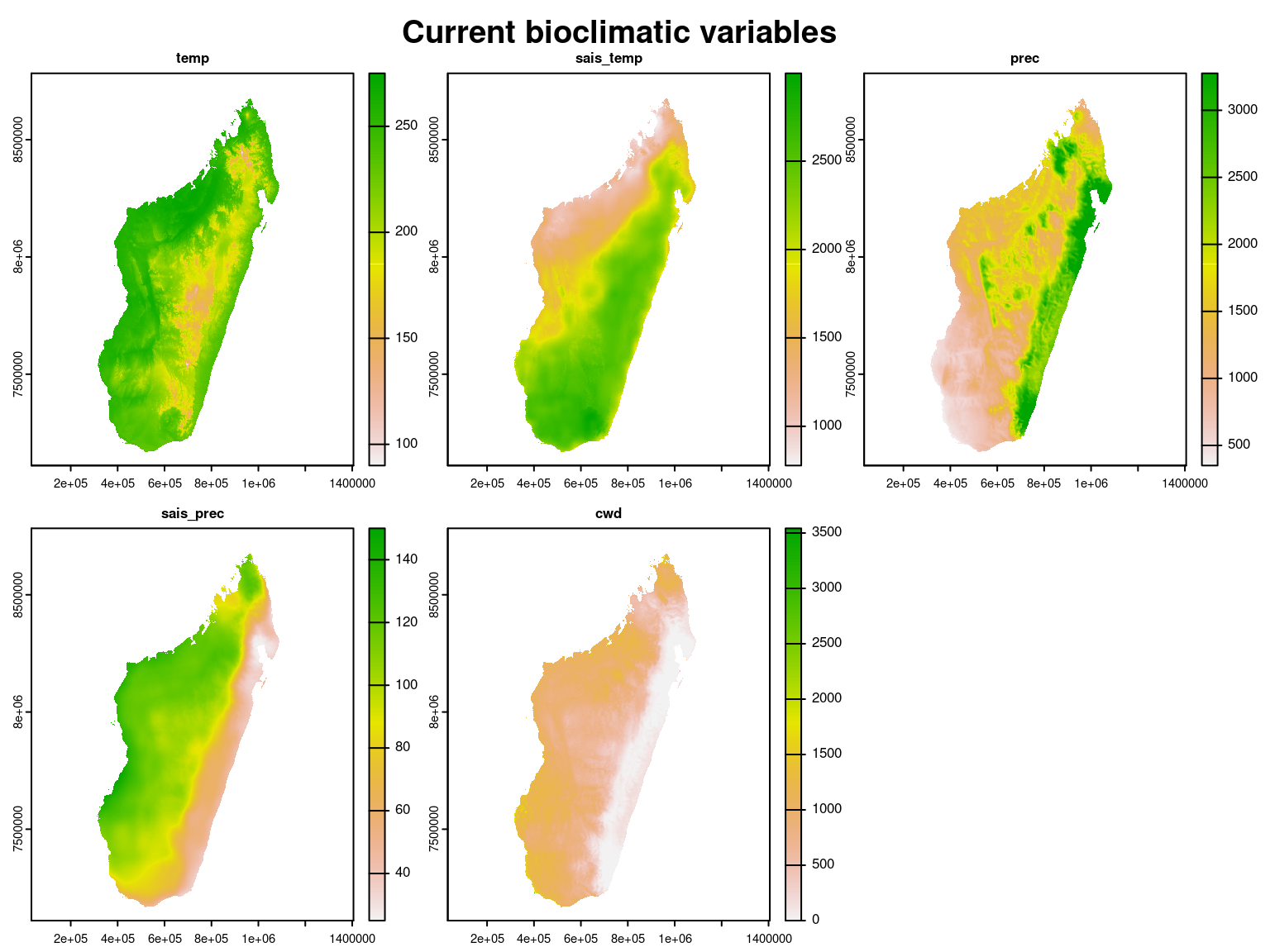The values of these climate variables corresponding to the coordinates of the inventory plots are extracted and scaled to obtain the following data-set where coordinates of the sites will then be used for spatial interpolation and to spatially represent the results.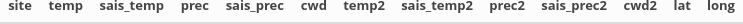## 3 Fitting joint species distribution model (JSDM) ### 3.1 Model definition Referring to the models used in the articles Warton et al. (2015) and Albert & Siddhartha (1993), we define the following latent variable model (LVM) to account for species co-occurrence on all sites : $\mathrm{probit}(\theta_{ij}) =\alpha_i + \beta_{0j}+X_i.\beta_j+ W_i.\lambda_j$ • Link function probit: $$\mathrm{probit}: q \rightarrow \Phi^{-1}(q)$$ where $$\Phi$$ correspond to the distribution function of the reduced centered normal distribution. • Response variable: $$Y=(y_{ij})^{i=1,\ldots, n_{site}}_{j=1,\ldots,n_{species}}$$ with: $y_{ij}=\begin{cases} 0 & \text{ if species j is absent on the site i}\\ 1 & \text{ if species j is present on the site i}. \end{cases}$ • Latent variable $$z_{ij} = \alpha_i + \beta_{0j} + X_i.\beta_j + W_i.\lambda_j + \epsilon_{i,j}$$, with $$\forall (i,j) \ \epsilon_{ij} \sim \mathcal{N}(0,1)$$ and such that: $y_{ij}=\begin{cases} 1 & \text{if} \ z_{ij} > 0 \\ 0 & \text{otherwise.} \end{cases}$ It can be easily shown that: $$y_{ij} \sim \mathcal{B}ernoulli(\theta_{ij})$$. • Latent variables: $$W_i=(W_i^1,\ldots,W_i^q)$$ where $$q$$ is the number of latent variables considered, which has to be fixed by the user (by default $$q=2$$). We assume that $$W_i \sim \mathcal{N}(0,I_q)$$ and we define the associated coefficients $$\lambda_j=(\lambda_j^1,\ldots, \lambda_j^q)'$$, also known as “factor loadings” . We use a prior distribution $$\mathcal{N}(0,1)$$ for all lambda not concerned by constraints to $$0$$ on upper diagonal and to strictly positive values on diagonal. • Explanatory variables: bioclimatic data about each site. $$X=(X_i)_{i=1,\ldots,n_{site}}$$ with $$X_i=(x_i^1,\ldots,x_i^p)\in \mathbb{R}^p$$ where $$p$$ is the number of bioclimatic variables considered. The corresponding regression coefficients for each species $$j$$ are noted : $$\beta_j=(\beta_j^1,\ldots,\beta_j^p)'$$. We use a prior distribution $$\mathcal{N}(0,1)$$ for all beta. • $$\beta_{0j}$$ correspond to the intercept for species $$j$$ which is assumed to be a fixed effect. We use a prior distribution $$\mathcal{N}(0,10)$$ for the species intercept. • $$\alpha_i$$ represents the random effect of site $$i$$ such as $$\alpha_i \sim \mathcal{N}(0,V_{\alpha})$$ and we assume that $$V_{\alpha} \sim \mathcal {IG}(\text{shape}=0.1, \text{rate}=0.1)$$ as prior distribution by default. This model is equivalent to a multivariate GLMM $$\mathrm{g}(\theta_{ij}) = \alpha_i + X_i.\beta_j + u_{ij}$$, where $$u_{ij} \sim \mathcal{N}(0, \Sigma)$$ with the constraint that the variance-covariance matrix $$\Sigma = \Lambda \Lambda^{\prime}$$, where $$\Lambda$$ is the full matrix of factor loadings, with the $$\lambda_j$$ as its columns. ### 3.2 Parameters inference We fit a binomial joint species distribution model, including two latent variables and random site effect using the jSDM_binomial_probit() function to perform binomial probit regression considering all the species from the data described above, by performing $$80 000$$ iterations including $$40 000$$ of burn-in and we retain $$N_{samp}=1 000$$ values for each parameter of the model. We use species’s prevalence as starting values for species intercept $$\beta_0$$. load("~/Documents/projet_BioSceneMada/Report/Internship_Report/data/scaled_data_clim.RData") data(madagascar, package="jSDM") PA <- madagascar # Prevalence of species np <- ncol(dplyr::select(scaled_data_clim2, -site, -lat, -long)) +1 prevalence <- colSums(PA)/nrow(PA) beta_start <- matrix(0,np,ncol(PA)) beta_start[1,] <- qnorm(prevalence) # T1<- Sys.time() mod_all <- jSDM::jSDM_binomial_probit( # Response variable presence_data = PA, # Explanatory variables site_formula = ~ temp + prec + sais_temp + sais_prec + cwd + temp2 + prec2 + sais_temp2 + sais_prec2 + cwd2, site_data=scaled_data_clim2, n_latent=2, site_effect="random", # Chains burnin= 40000, mcmc=40000, thin=40, # Starting values alpha_start=0, beta_start=beta_start, lambda_start=0, W_start=0, V_alpha=1, # Priors shape_Valpha=0.1, rate_Valpha=0.1, mu_beta=0, V_beta=c(10,rep(1,np-1)), mu_lambda=0, V_lambda=1, # Various seed=1234, verbose=1) T2 <- Sys.time() T_all <- difftime(T2,T1) save(mod_all,T_all,file="~/Documents/projet_BioSceneMada/Report/Internship_Report/data/mada_mod.RData")The MCMC algorithm is used to obtain draws from the posterior distribution of the parameters. We use as estimator for each parameter the mean of the $$N_{samp}$$ values estimated in corresponding MCMC chain and we save the estimated parameters in .csv format. load("~/Documents/projet_BioSceneMada/Report/Internship_Report/data/mada_mod.RData") load("~/Documents/projet_BioSceneMada/Report/Internship_Report/data/scaled_data_clim.RData") species <- colnames(mod_all$model_spec$presence_data) nplot <- nrow(mod_all$model_spec$presence_data) nsp <- ncol(mod_all$model_spec$presence_data) n_latent <- mod_all$model_spec$n_latent np <- nrow(mod_all$model_spec$beta_start) ## Save parameters ### alphas alphas <- apply(mod_all$mcmc.alpha,2,mean)
### V_alpha
V_alpha <- mean(mod_all$mcmc.V_alpha) ### latent variables W1 <- colMeans(mod_all$mcmc.latent[["lv_1"]])
W2 <- colMeans(mod_all$mcmc.latent[["lv_2"]]) params_sites <- data.frame(plot = scaled_data_clim2$site,
lat = scaled_data_clim2$lat, long = scaled_data_clim2$long,
alphas, V_alpha = rep(V_alpha,nplot),W1,W2)
write.csv(params_sites, file = "~/Documents/projet_BioSceneMada/Report/Internship_Report/data/params_sites.csv",row.names = F)

### fixed species effect lambdas and betas
lambdas <- matrix(0,nsp,n_latent)
betas <- matrix(0,nsp,np)

for (j in 1:nsp){
for (l in 1:n_latent){
lambdas[j,l] <- mean(mod_all$mcmc.sp[[j]][,np+l]) } for (p in 1:np){ betas[j,p] <- mean(mod_all$mcmc.sp[[j]][,p])
}
}
colnames(betas) <- colnames(mod_all$mcmc.sp[])[1:np] params_species <- data.frame(species=species, Id_species = c(1:nsp), betas, lambda_1 = lambdas[,1], lambda_2 = lambdas[,2]) write.csv(params_species, file ="~/Documents/projet_BioSceneMada/Report/Internship_Report/data/params_species.csv", row.names = F) ## probit_theta_latent write.csv(mod_all$probit_theta_latent,
row.names = F)
## theta_latent
write.csv(mod_all$theta_latent, file = "~/Documents/projet_BioSceneMada/Report/Internship_Report/data/theta_latent.csv", row.names = F) ### 3.3 Evaluation of MCMC convergence We visually evaluate the convergence of MCMCs by representing the trace and density a posteriori of the estimated parameters. load("~/Documents/projet_BioSceneMada/Report/Internship_Report/data/mada_mod.RData") np <- nrow(mod_all$model_spec$beta_start) species <- colnames(mod_all$model_spec$presence_data) ## alpha_i of the first site par(mfrow=c(1,2),oma=c(1, 0, 1.4, 0)) coda::traceplot(mod_all$mcmc.alpha[,1],main="Trace of alpha_1",cex.main=1.6)
coda::densplot(mod_all$mcmc.alpha[,1],main="Density of alpha_1", cex.main=1.6) abline(v=mean(mod_all$mcmc.alpha[,1]),col="blue")
title(main="Random site effect alpha_1",outer=T,cex.main=1.8)
## V_alpha
coda::traceplot(mod_all$mcmc.V_alpha,main="Trace of V_alpha",cex.main=1.6) coda::densplot(mod_all$mcmc.V_alpha,main="Density of V_alpha", cex.main=1.6)
abline(v=mean(mod_all$mcmc.V_alpha),col="blue") title(main=" Variance of random site effects",outer=T, cex.main=1.8) ## beta_j of the first two species for (j in 1:2) { par(mfrow=c(2,2),oma=c(1, 0, 1.4, 0)) for (p in 1:np) { coda::traceplot(mod_all$mcmc.sp[[j]][,p],
main=paste("Trace of", colnames(mod_all$mcmc.sp[[j]])[p]),cex.main=1.3) coda::densplot(mod_all$mcmc.sp[[j]][,p],
main=paste("Density of", colnames(mod_all$mcmc.sp[[j]])[p]),cex.main=1.3) abline(v=mean(mod_all$mcmc.sp[[j]][,p]),col="blue")
if(p==1) title(main=paste("Fixed species effect beta for species", species[j]), outer=T, cex.main=1.6)
}
}

## lambda_j of the first two species
n_latent <- mod_all$model_spec$n_latent
par(mfrow=c(n_latent,2),oma=c(1, 0, 1.4, 0))
for (j in 1:2) {
for (l in 1:n_latent) {
coda::traceplot(mod_all$mcmc.sp[[j]][,np+l], main = paste("Trace of", colnames(mod_all$mcmc.sp[[j]])[np+l]),cex.main=1.6)
coda::densplot(mod_all$mcmc.sp[[j]][,np+l], main = paste("Density of", colnames(mod_all$mcmc.sp[[j]])[np+l]),cex.main=1.6)
abline(v=mean(mod_all$mcmc.sp[[j]][,np+l]),col="blue") } title(main=paste("Factor loadings lambda for species ", species[j]),outer=T,cex.main=1.8) } ## Latent variables W_i for one site par(mfrow=c(2,2),oma=c(1, 0, 1.4, 0)) for (l in 1:n_latent) { coda::traceplot(mod_all$mcmc.latent[[paste0("lv_",l)]][,1],
main = paste0(" Trace of W_1", l),cex.main=1.6)
coda::densplot(mod_all$mcmc.latent[[paste0("lv_",l)]][,1], main = paste0(" Density of W_1", l),cex.main=1.6) abline(v=mean(mod_all$mcmc.latent[[paste0("lv_",l)]][,1]),col="blue")
}
title(main="Latentes variables W_1", outer=T,cex.main=1.8)
## Deviance
par(mfrow=c(1,2),oma=c(1, 0, 1.5, 0))
coda::traceplot(mod_all$mcmc.Deviance,main="Trace",cex.main=1.6) coda::densplot(mod_all$mcmc.Deviance,main="Density",cex.main=1.6)
abline(v=mean(mod_all$mcmc.Deviance),col="blue") title(main = "Deviance",outer=T,cex.main=1.8) # theta par(mfrow=c(1,2)) hist(mod_all$probit_theta_latent,col="light blue",  main = "probit(theta) estimated")
species_richness$species_richness, main="Species richness", xlab="observed", ylab="estimated", cex.main=1.4, cex.lab=1.3) abline(a=0,b=1,col='red')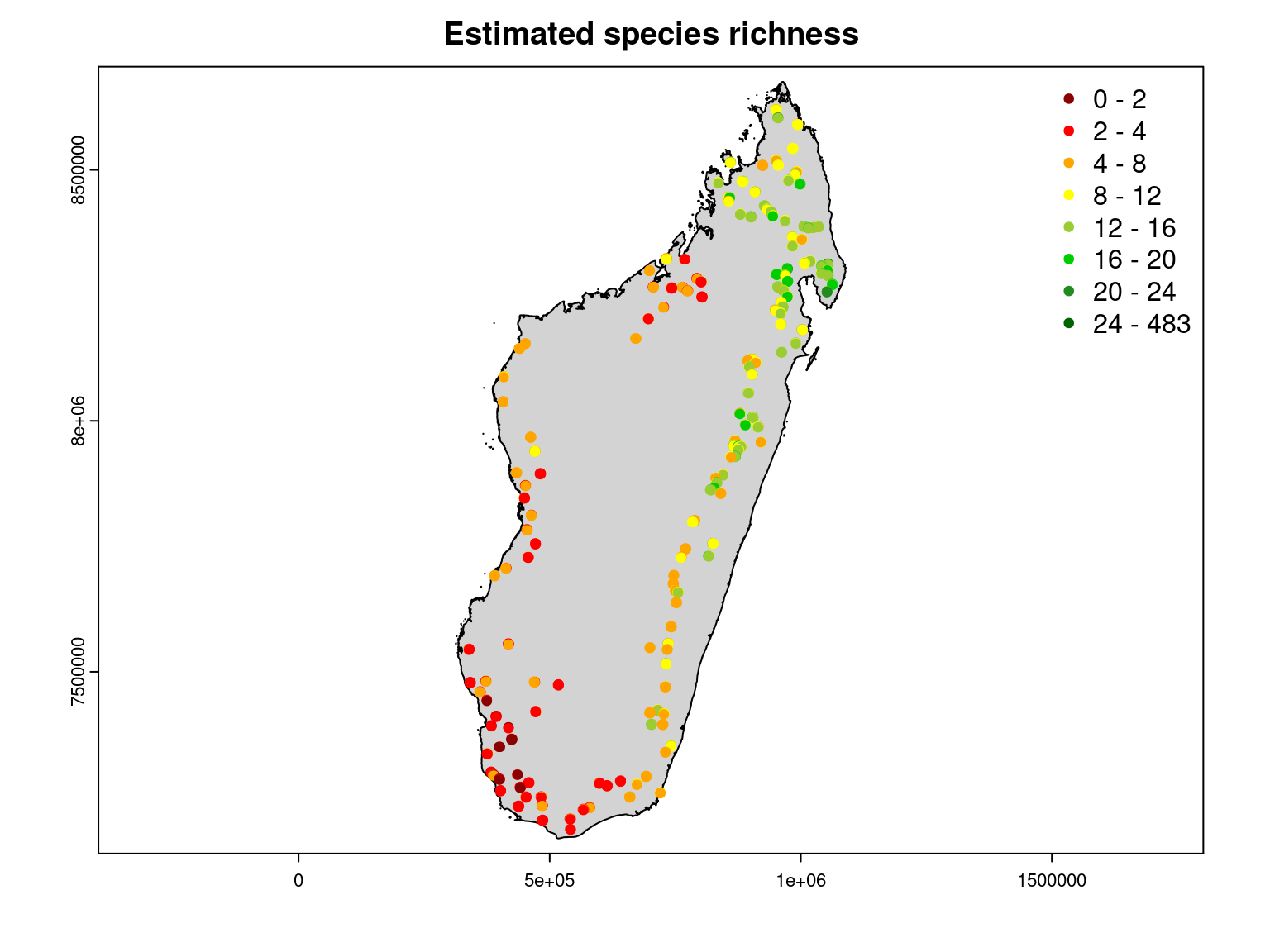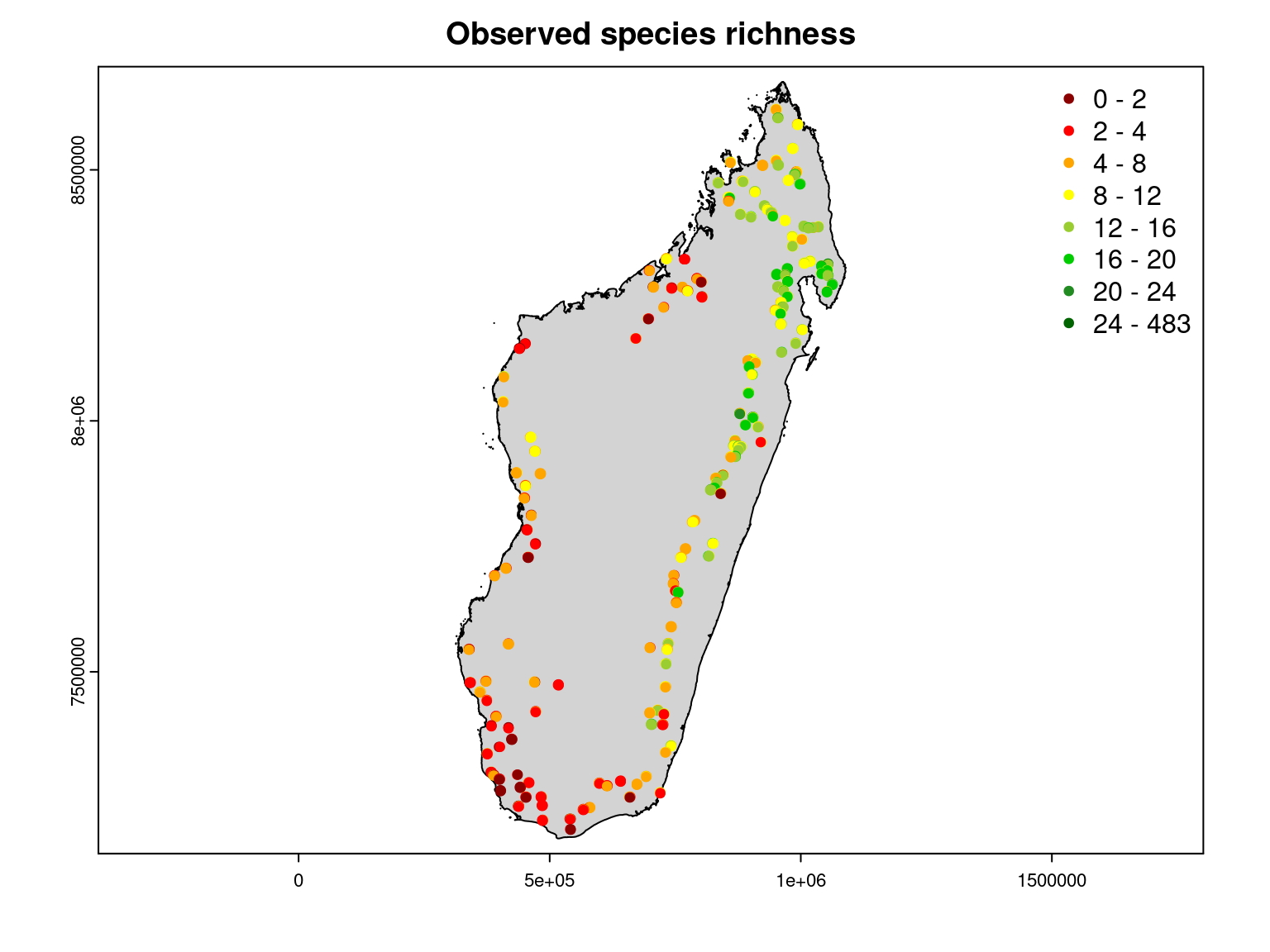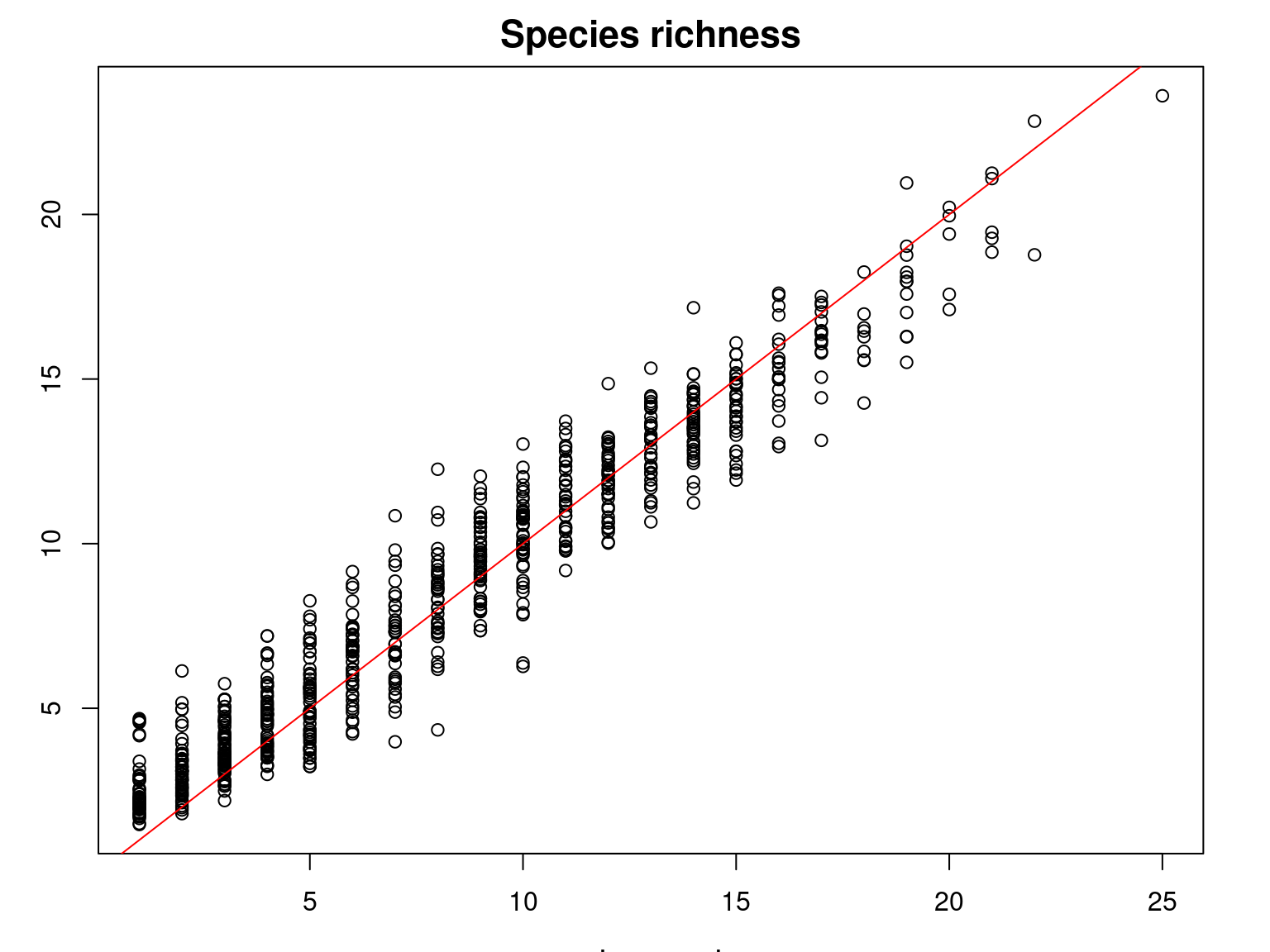It can be seen that the observed species richness on the inventory sites corresponds fairly well to that estimated by summing the estimated probabilities of occurrence of all species on each site. Moreover, we can see that the observed species richness is greater in the moist forest of the north-east of the island, particularly in the Masoala peninsula. ### 4.3 Residual correlation After fitting the JSDM with latent variables, the full species residual correlation matrix $$R=(R_{ij})^{i=1,\ldots, n_{site}}_{j=1,\ldots, n_{species}}$$ can be derived from the covariance in the latent variables such as : $\Sigma_{ij} = \lambda_i^T .\lambda_j$, then we compute correlations from covariances : $R_{i,j} = \frac{\Sigma_{ij}}{\sqrt{\Sigma _{ii}\Sigma _{jj}}}$. load("~/Documents/projet_BioSceneMada/Report/Internship_Report/data/mada_mod.RData") n.species <- ncol(mod_all$model_spec$presence_data) n.mcmc <- nrow(mod_all$mcmc.latent[])
Tau.cor.arr <- matrix(NA,n.mcmc,n.species^2)

for(t in 1:n.mcmc) {
lv.coefs <- t(sapply(mod_all$mcmc.sp, "[", t, grep("lambda",colnames(mod_all$mcmc.sp[]))))
Tau.mat <- lv.coefs %*% t(lv.coefs)
Tau.cor.mat <- cov2cor(Tau.mat)
Tau.cor.arr[t,] <- as.vector(Tau.cor.mat)
}
## Average over the MCMC samples
R <- matrix(apply(Tau.cor.arr,2,mean),n.species,byrow=F)
write.csv(R, file ="~/Documents/projet_BioSceneMada/Report/Internship_Report/data/R.csv", row.names = F)

We plot the top 50 estimated residual correlations between species based on their number of observed occurrences and environmental preferences.

# Forest inventory
# Species parameters
# Residual correlation matrix R
colnames(R) <- rownames(R) <- make.names(params_species$species) R <- as.matrix(R) library(viridis) jSDM::plot_associations(R, circleBreak=TRUE, occ=PA, top=50, main="Species-species association sorted after their frequency", cols_association=viridis::viridis(10), cols_occurrence=viridis::magma(10), species_order="frequency") # Average of MCMC samples of species effect beta except the intercept effects = params_species[,grep("beta", colnames(params_species))[-1]] np <- ncol(effects) # Add absolute quadratic and linear effects of each environmental variable effects <- abs(effects[,1:(np/2)]) + abs(effects[,(np/2 +1):np]) colnames(effects) <- gsub("beta_", "",colnames(effects)) jSDM::plot_associations(R, env_effect=effects, top=50, main="Species-species association sorted after their main environmental effect", cols_association=viridis::viridis(10), species_order="main env_effect")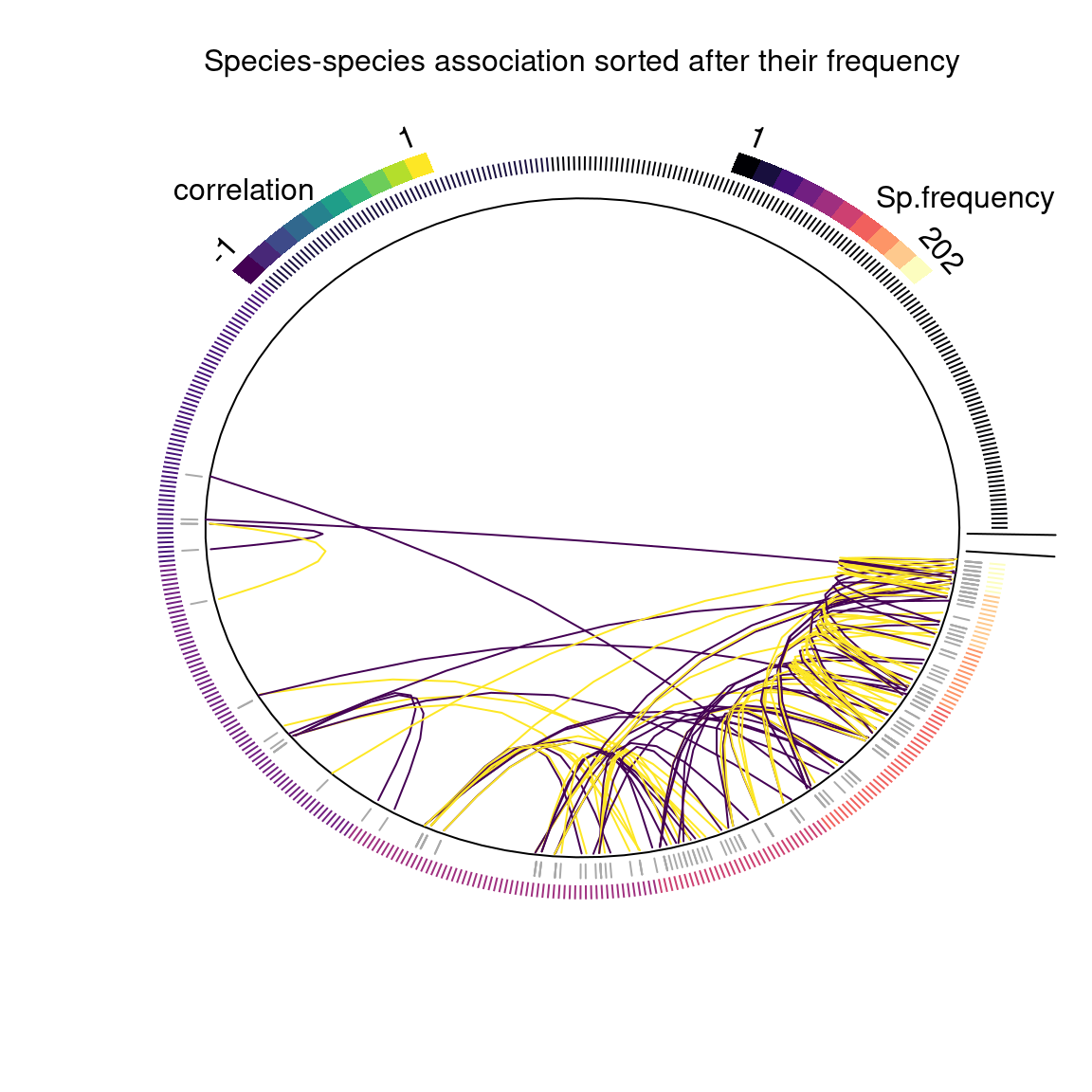The figure above shows the correlations between species, with the 483 species sorted according to the number of sites where they occur out of the 751 inventoried.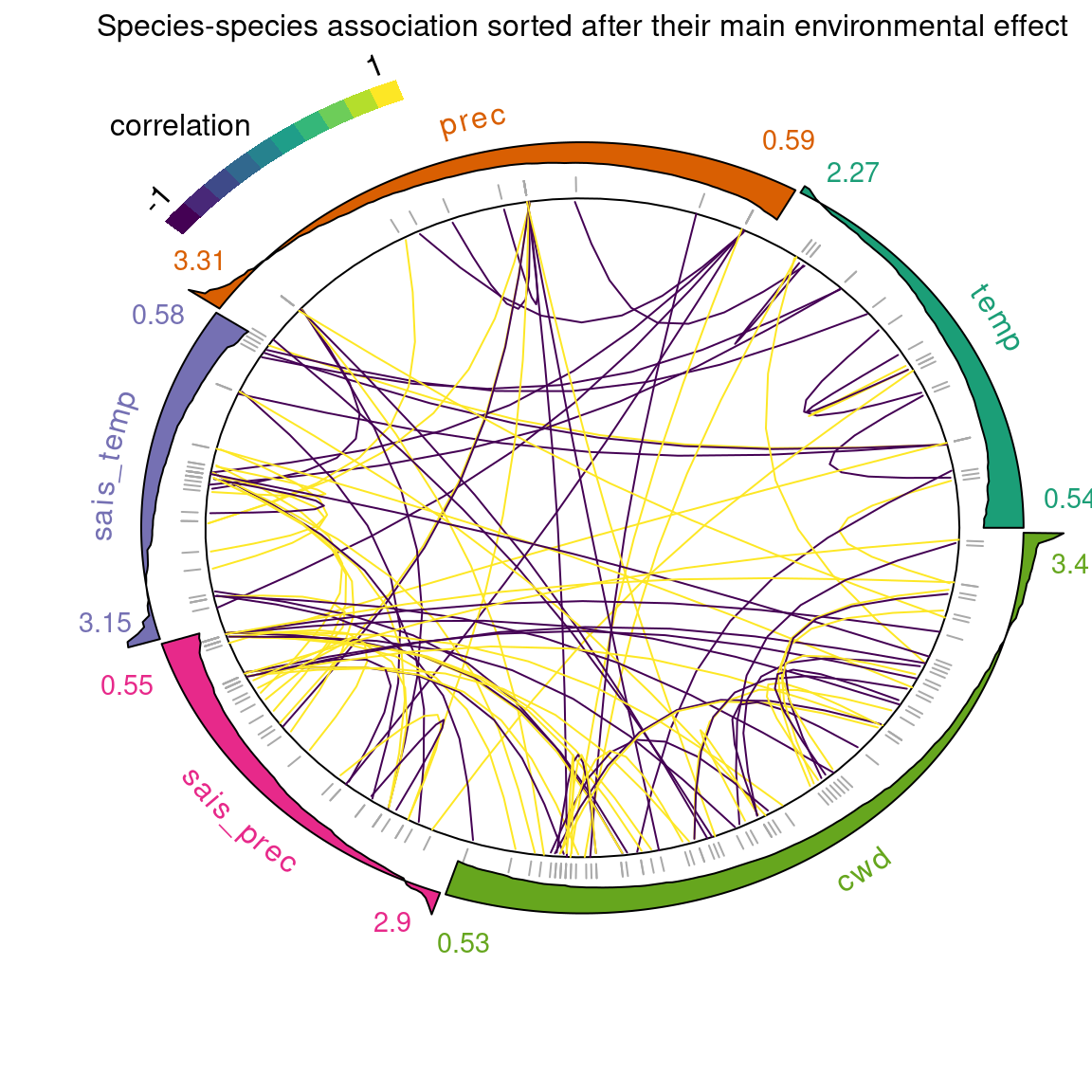The figure above shows the same covariance structure but with the species sorted according to their largest environmental coefficients ($$\beta$$) (the outer ring shows the distribution of environmental effect for species within the sample). This representation of associations between species allows us to observe the positive or negative correlations between species which can be interpreted in terms of positive or negative influence of the presence of a species on the probability of occurrence of another. ## 5 Intrapolation of parameters between inventory sites The presence of a spatial structure where observations close to each other are more similar than those far away (spatial auto-correlation) is a prerequisite for the application of geostatistics and from the previous spatial representations it seems to be satisfied. Thus, it is possible to interpolate sites’ parameters for the whole island from those estimated for the inventory plots using the spatial interpolation method called regularized spline with tension (RST) from GRASS GIS software via rgrass7 R package. This method is described in the article . ### 5.1 Random site effect $$\alpha$$ ## Initialize GRASS setwd("~/Documents/jSDM/vignettes") Sys.setenv(LD_LIBRARY_PATH=paste("/usr/lib/grass78/lib", Sys.getenv("LD_LIBRARY_PATH"),sep=":")) library(rgrass7) # use a georeferenced raster system('grass -c ~/Documents/projet_BioSceneMada/Report/Internship_Report/data/scaled_clim.tif grassdata/interpolation') # connect to grass database initGRASS(gisBase="/usr/lib/grass78", gisDbase="grassdata", location="interpolation", mapset="PERMANENT", override=TRUE) We interpolate the random site effect at Madagascar scale using RST method. library(rgrass7) # Import sites parameters params_sites <- read.csv("~/Documents/projet_BioSceneMada/Report/Internship_Report/data/params_sites.csv") # inventory sites coordinates in UTM38S projection xy <- terra::vect("/home/clement/Documents/projet_BioSceneMada/Report/Internship_Report/data/coords.shp") # alpha alpha_sp <- terra::vect(data.frame(alpha=params_sites$alphas, terra::crds(xy)),
geom=c("x","y"), crs=terra::crs(xy))
rgrass7::write_VECT(alpha_sp, "alpha", flags="overwrite")
# Re-sample with RST
# for punctual data use function v.surf.rst
system("v.surf.rst --overwrite --verbose -t tension=3 input=alpha zcolumn=alpha \\
smooth=0.0 elevation=alpha_rst")
# Export
system("r.out.gdal --overwrite input=alpha_rst \\
createopt='compress=lzw,predictor=2'")
# Representation
# Data restricted to Madagascar's borders
borders <- terra::project(borders, terra::crs(alpha_rst))
# terra::writeRaster(alpha_rst,
#                    gdal=c("COMPRESS=LZW", "PREDICTOR=2"), overwrite=TRUE)
terra::plot(alpha_rst, main="Site effect alpha interpolated by RST")
alpha_rst_xy <- terra::extract(alpha_rst, xy)[,"alpha_rst"]
plot(alpha_rst_xy, params_sites$alphas, xlab="alpha interpolated by RST", ylab="alpha estimated by JSDM", main="Random site effect") abline(a=0, b=1, col='red') # Center interpolated site effect alpha_rst_centered <- terra::app(alpha_rst, fun=scale, scale=FALSE) names(alpha_rst_centered) <- names(alpha_rst) terra::plot(alpha_rst_centered, main="Site effect alpha interpolated by RST") alpha_rst_centered_xy <- terra::extract(alpha_rst_centered, xy)[,"alpha_rst"] plot(alpha_rst_centered_xy, params_sites$alphas,
xlab="alpha interpolated by RST",
ylab="alpha estimated by JSDM",
main="Random site effect")
abline(a=0, b=1, col='red')
gdal=c("COMPRESS=LZW", "PREDICTOR=2"), overwrite=TRUE)

We represent the random site effect interpolated at Madagascar scale.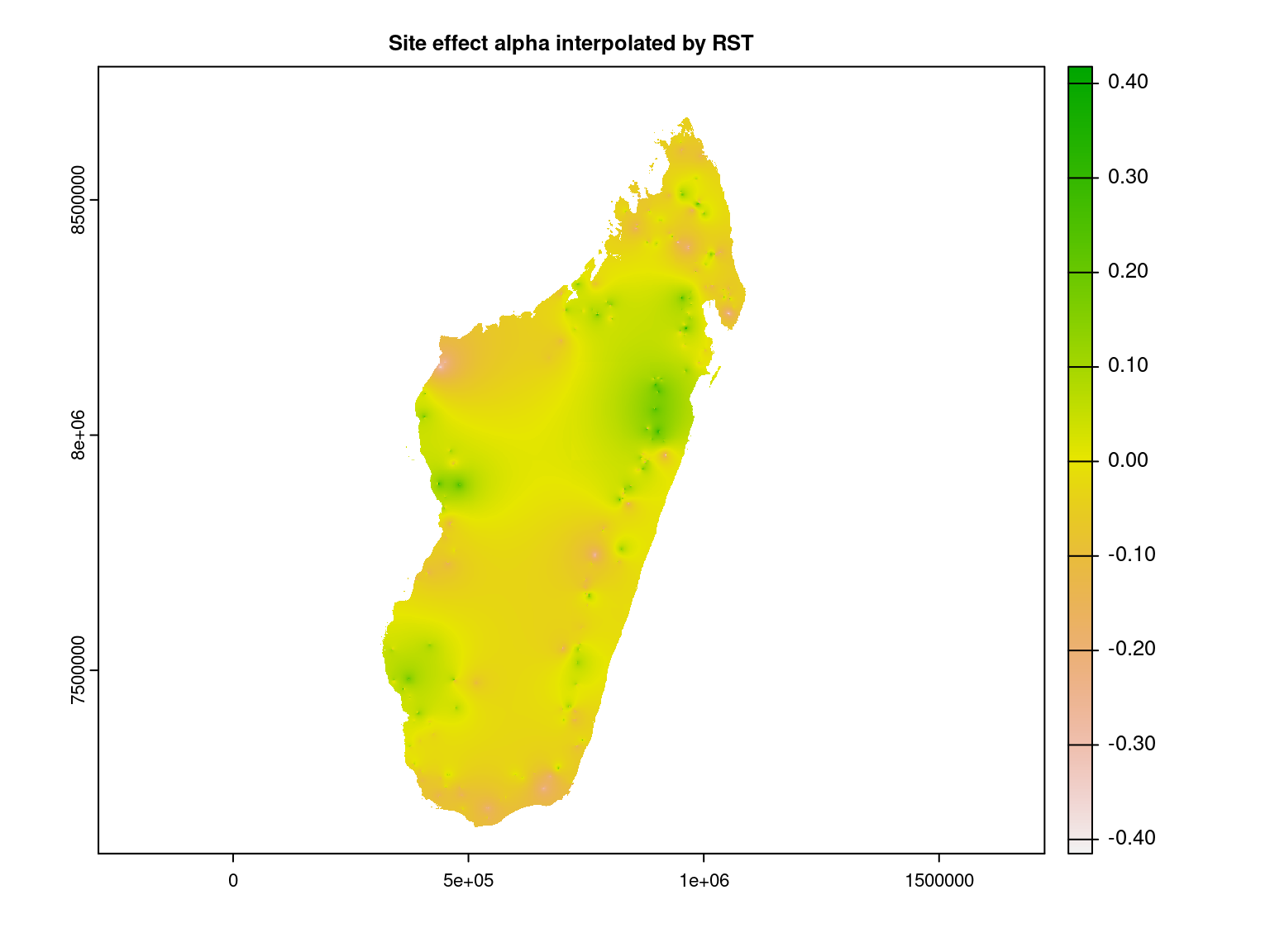### 5.2 Latent variables $$W$$

We interpolate the first axis of latent variables at the Madagascar scale using the RST method.

# W1
W1_sp <- terra::vect(data.frame(W1=params_sites$W1, terra::crds(xy)), geom=c("x","y"), crs=terra::crs(xy)) rgrass7::write_VECT(W1_sp, "W1", flags="overwrite") # Re-sample with RST # Note: use mask=Madagascar to save computation time # for punctual data use function v.surf.rst system("v.surf.rst --overwrite --verbose -t tension=3 input=W1 zcolumn=W1 \\ smooth=0.0 elevation=W1_rst") # Export system("r.out.gdal --overwrite input=W1_rst \\ output=Madagascar_cache/W1_rst.tif type=Float32 \\ createopt='compress=lzw,predictor=2'") # Representation W1_rst <- terra::rast("Madagascar_cache/W1_rst.tif") # Data restricted to Madagascar's borders borders <- terra::vect(output[], layer="gadm36_MDG_0") borders <- terra::project(borders, terra::crs(W1_rst)) W1_rst <- terra::mask(W1_rst, borders) terra::plot(W1_rst, main="Latent variable W1 interpolated by RST") W1_rst_xy <- terra::extract(W1_rst, xy)[, "W1_rst"] plot(W1_rst_xy, params_sites$W1,
xlab="W1 interpolated by RST",
ylab="W1 estimated by JSDM",
main="First latent axis")
abline(a=0, b=1, col='red')

# Center interpolated first latent axis
W1_rst_centered <- terra::app(W1_rst, scale, scale=FALSE)
names(W1_rst_centered) <- names(W1_rst)
terra::plot(W1_rst_centered, main="First latent axis interpolated by RST")
W1_rst_centered_xy <- terra::extract(W1_rst_centered, xy)[,"W1_rst"]
plot(W1_rst_centered_xy, params_sites$W1, xlab="W1 interpolated by RST", ylab="W1 estimated by JSDM", main="First latent axis") abline(a=0, b=1, col='red') terra::writeRaster(W1_rst_centered, "Madagascar_cache/W1_rst_centered.tif", gdal=c("COMPRESS=LZW", "PREDICTOR=2"), overwrite=TRUE) We represent the first latent variable interpolated at Madagascar scale.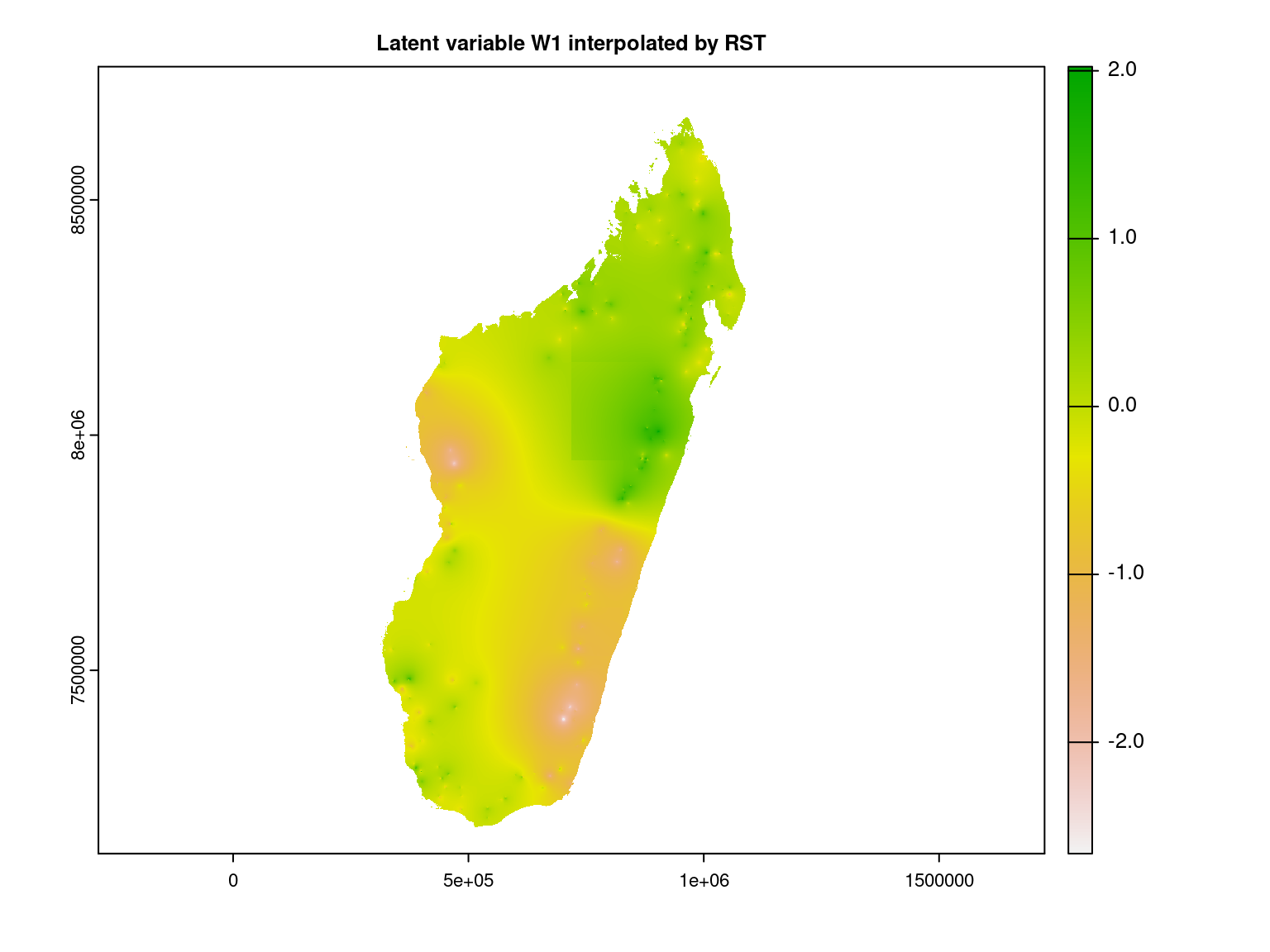We interpolate the second axis of latent variables at the Madagascar scale using the RST method. # W2 W2_sp <- terra::vect(data.frame(W2=params_sites$W2, terra::crds(xy)),
geom=c("x","y"), crs=terra::crs(xy))
rgrass7::write_VECT(W2_sp, "W2", flags="overwrite")
# Re-sample with RST
# for punctual data use function v.surf.rst
system("v.surf.rst --overwrite --verbose -t tension=3 input=W2 zcolumn=W2 \\
smooth=0.0 elevation=W2_rst")
# Export
system("r.out.gdal --overwrite input=W2_rst \\
createopt='compress=lzw,predictor=2'")
# Representation
# Data restricted to Madagascar's borders
borders <- terra::project(borders, terra::crs(W2_rst))
terra::plot(W2_rst, main="Latent variable W2 interpolated by RST")
W2_rst_xy <- terra::extract(W2_rst, xy)[, "W2_rst"]
plot(W2_rst_xy, params_sites$W2, xlab="W2 interpolated by RST", ylab="W2 estimated by JSDM", main="Second latent axis") abline(a=0, b=1, col='red') # Center interpolated first latent axis W2_rst_centered <- terra::app(W2_rst, scale, scale=FALSE) names(W2_rst_centered) <- names(W2_rst) terra::plot(W2_rst_centered, main="Second latent axis interpolated by RST") W2_rst_centered_xy <- terra::extract(W2_rst_centered, xy)[,"W2_rst"] plot(W2_rst_centered_xy, params_sites$W2,
xlab="W2 interpolated by RST",
ylab="W2 estimated by JSDM",
main="Second latent axis")
abline(a=0, b=1, col='red')
gdal=c("COMPRESS=LZW", "PREDICTOR=2"), overwrite=TRUE)

We represent the second latent variable interpolated at Madagascar scale.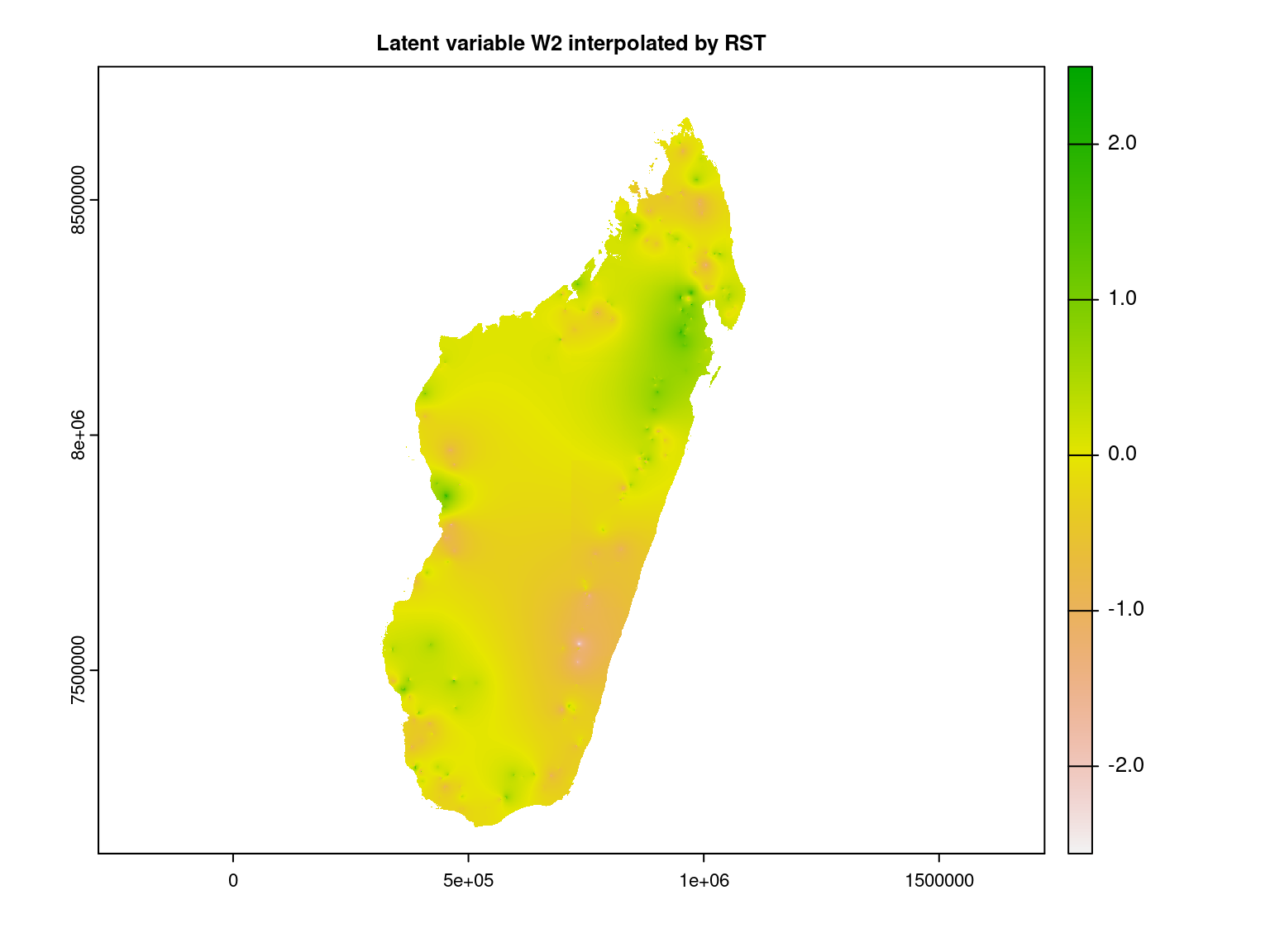## 6 Representation of results at the Madagascar scale

### 6.1 Predictives maps of presence probabilities

We calculate the probabilities of species presences between inventory sites using interpolated site’s parameters.

remove(list = ls())
# RST interpolation of sites parameters

# Species parameters
nsp <- nrow(params_species)
# Current climatic variables
names(scaled_clim_var) <- colnames(X[,-1])
terra::ext(scaled_clim_var) <- terra::ext(rst_alpha)
if(terra::crs(scaled_clim_var) != terra::crs(rst_alpha)){
scaled_clim_var <- terra::project(scaled_clim_var, terra::crs(rst_alpha))
}

# Function to compute probit_theta at Madagascar scale
predfun <- function(scaled_clim_var, params_species, rst_alpha, rst_W1, rst_W2, species.range){
lambda_1 <- as.matrix(params_species[,"lambda_1"])
lambda_2 <- as.matrix( params_species[,"lambda_2"])
beta <- as.matrix(params_species[,3:13])

## Xbeta_1
np <- terra::nlyr(scaled_clim_var)
Xbeta_1 <- terra::rast(ncols=dim(rst_alpha), nrows=dim(rst_alpha),
ext=terra::ext(rst_alpha), crs=terra::crs(rst_alpha),
resolution=terra::res(rst_alpha))
terra::values(Xbeta_1) <- rep(beta[1,1][], terra::ncell(Xbeta_1))
for (p in 1:np) {
Xbeta_1 <- Xbeta_1 + scaled_clim_var[[p]]*beta[1,p+1]
}
## Wlambda_1
Wlambda_1 <- rst_W1*lambda_1 + rst_W2*lambda_2
## probit_theta_1
probit_theta_1 <- Xbeta_1 + Wlambda_1 + rst_alpha
probit_theta <- probit_theta_1
remove(list=c("probit_theta_1","Wlambda_1"))
## Other species
for (j in (species.range+1):species.range) {
## Xbeta_j
Xbeta_j <- Xbeta_1
terra::values(Xbeta_j) <- rep(beta[j,1][], terra::ncell(Xbeta_j))
for (p in 1:np) {
Xbeta_j <- Xbeta_j + scaled_clim_var[[p]]*beta[j,p+1]
}
## Wlambda_j
Wlambda_j <- rst_W1*lambda_1[j] + rst_W2*lambda_2[j]
## probit_theta_j
probit_theta_j <- Xbeta_j + Wlambda_j + rst_alpha
probit_theta <- c(probit_theta, probit_theta_j)
remove(list=c("probit_theta_j", "Xbeta_j", "Wlambda_j"))
}
names(probit_theta) <- make.names(params_species$species[species.range:species.range]) return(probit_theta) } # Compute theta in ten parts because it's too large npart <- 10 first.species <- seq(1, nsp, by=floor(nsp/npart)+1) for (n in 1:npart){ probit_theta <- predfun(scaled_clim_var, params_species, rst_alpha, rst_W1, rst_W2, species.range=c(first.species[n], min(nsp,first.species[n]+floor(nsp/npart)))) terra::writeRaster(probit_theta, filename=paste0("~/Documents/projet_BioSceneMada/Report/Internship_Report/data/RST_centered_probit_theta_", n, ".tif"), filetype="GTiff", gdal=c("COMPRESS=LZW", "PREDICTOR=2"), overwrite=TRUE) # Compute and save SpatRaster of probabilities of presence theta terra::app(probit_theta, pnorm, cores=2, filename=paste0("~/Documents/projet_BioSceneMada/Report/Internship_Report/data/RST_centered_theta_",n,".tif"), overwrite=TRUE, wopt=list(gdal=c("COMPRESS=LZW", "PREDICTOR=2"), filetype="GTiff")) remove(probit_theta) } We represent the presence probabilities of two species interpolated at the scale of Madagascar as well as their observed occurrences at the inventory sites represented by black crosses. params_species <- read.csv(file = "~/Documents/projet_BioSceneMada/Report/Internship_Report/data/params_species.csv") nsp <- nrow(params_species) xy <- terra::vect("/home/clement/Documents/projet_BioSceneMada/Report/Internship_Report/data/coords.shp") data(madagascar, package="jSDM") PA <- madagascar # Observed presence absence id_pres_Ocotea <- which(PA[,"sp_1"]==1) obs_pres_Ocotea <- terra::vect(terra::crds(xy[id_pres_Ocotea,]), type="point", crs=terra::crs(xy)) id_pres_Manilkara <- which(PA[,"sp_6"]==1) obs_pres_Manilkara <- terra::vect(terra::crds(xy[id_pres_Manilkara,]), type="point", crs=terra::crs(xy)) # Representation npart <- 10 first.species <- seq(1,nsp,by=floor(nsp/npart)+1) theta <- terra::rast("~/Documents/projet_BioSceneMada/Report/Internship_Report/data/RST_theta_1.tif") names(theta) <- paste0("sp_", first.species:(first.species+floor(nsp/npart))) par(mfrow=c(1,2), oma=c(1, 0, 2, 1), mar=c(5, 4, 3, 2)) terra::plot(theta$sp_1,
main="Ocotea.laevis")
terra::points(obs_pres_Ocotea, pch=3, cex=0.7)
terra::plot(theta$sp_6, main="Manilkara.hexandra") terra::points(obs_pres_Manilkara, pch=3, cex=0.7) title(main="Interpolated current probabilities of presence", outer=T, cex.main=1.3)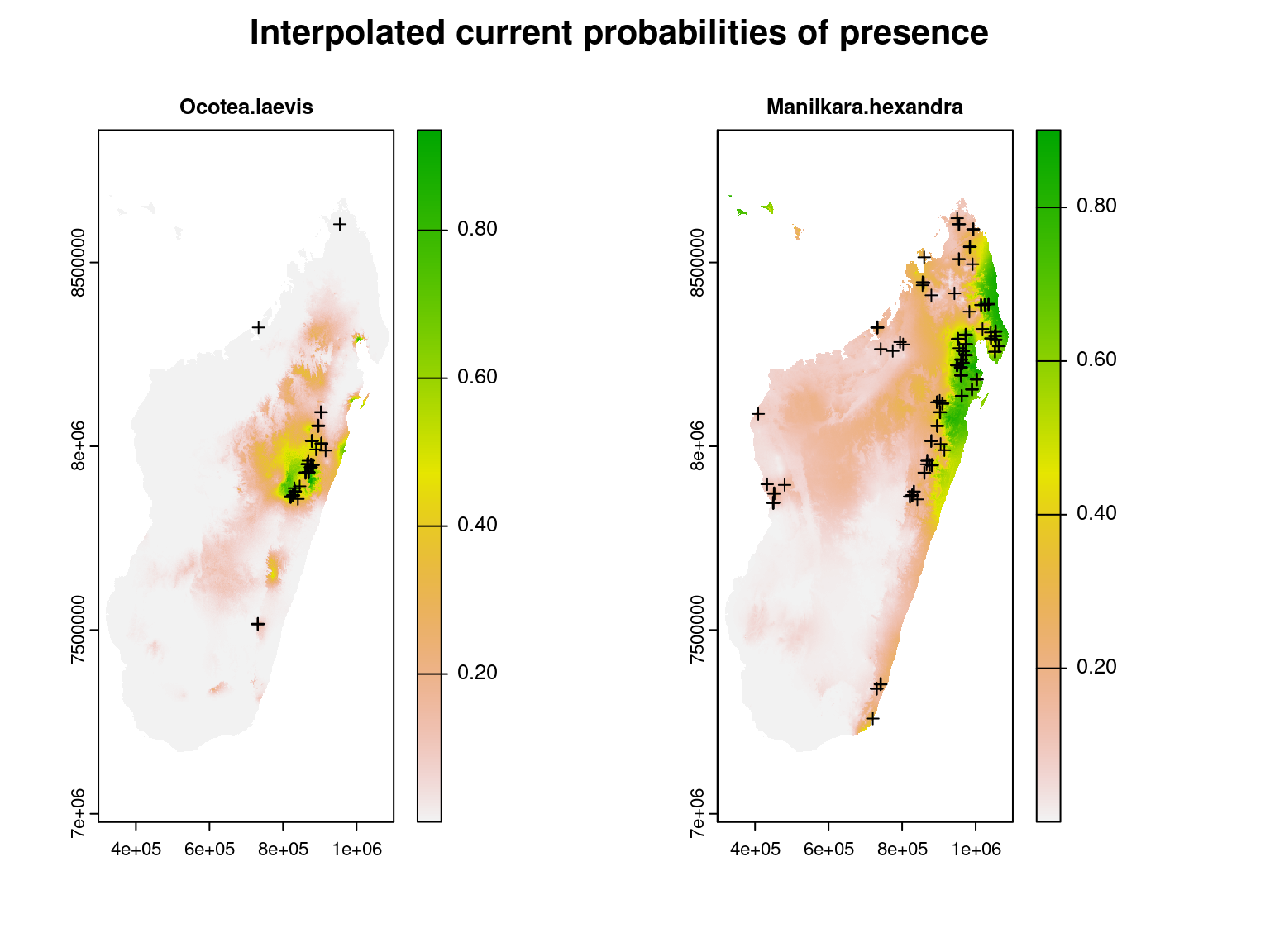We can see that the areas with high estimated probabilities of occurrence for these species correspond well to the inventory sites where these species have been observed. ### 6.2 Predictive maps of species richness (diversity $$\alpha$$) Species richness also called diversity $$\alpha$$ reflects the number of species coexisting in a given environment and is computed by summing the number of species present on each site. #### 6.2.1 Current species richness We compute at Madagascar scale the species richness by summing the interpolated probabilities of occurrence for each map’s cell $$i$$ : $$\widehat{R}_i=\sum\limits_ {j=1}^{n_{species}} \widehat{\theta}_{ij}$$. theta <- terra::rast("~/Documents/projet_BioSceneMada/Report/Internship_Report/data/RST_theta_1.tif") species_richness <- terra::app(theta, sum) npart <- 10 for(n in 2:npart){ theta <- terra::rast(paste0("~/Documents/projet_BioSceneMada/Report/Internship_Report/data/RST_theta_", n, ".tif")) species_richness <- species_richness + terra::app(theta, sum) } names(species_richness) <- "species_richness" terra::writeRaster(species_richness, filename=paste0("~/Documents/projet_BioSceneMada/Report/Internship_Report/data/species_richness.tif"), filetype="GTiff", gdal=c("COMPRESS=LZW", "PREDICTOR=2"), overwrite=TRUE) #### 6.2.2 Current species richness restricted to forest cover However, the model used does not take into account human presence, which is manifested in particular by the deforestation of the island, so we use data on remaining forest cover in 2000 from the article Vieilledent et al. (2018) in order to replace with zero values the interpolated species richness at locations where forest is known to have been removed. library(glue) # raster of Madagascar's forest cover in 2000 forest <- terra::rast("~/Documents/projet_BioSceneMada/Report/Internship_Report/data/for2000.tif") # change resolution of species richness raster from 1000x1000m to 30x30m in_f <- "~/Documents/projet_BioSceneMada/Report/Internship_Report/data/species_richness.tif" out_f <- "~/Documents/projet_BioSceneMada/Report/Internship_Report/data/species_richness_30m.tif" cmd <- glue('gdalwarp -tr 30 30 -te {xmin(forest)} {ymin(forest)} {xmax(forest)} {ymax(forest)} -r near -co "COMPRESS=LZW" -co "PREDICTOR=2" -overwrite {in_f} {out_f}') system(cmd) # remove species richness values where there was no forest in 2000s species_richness <- terra::rast("~/Documents/projet_BioSceneMada/Report/Internship_Report/data/species_richness_30m.tif") species_richness_deforest <- terra::mask(species_richness, forest) terra::writeRaster(species_richness_deforest, filename="~/Documents/projet_BioSceneMada/Report/Internship_Report/data/species_richness_deforest.tif", filetype="GTiff", gdal=c("COMPRESS=LZW", "PREDICTOR=2"), overwrite=TRUE)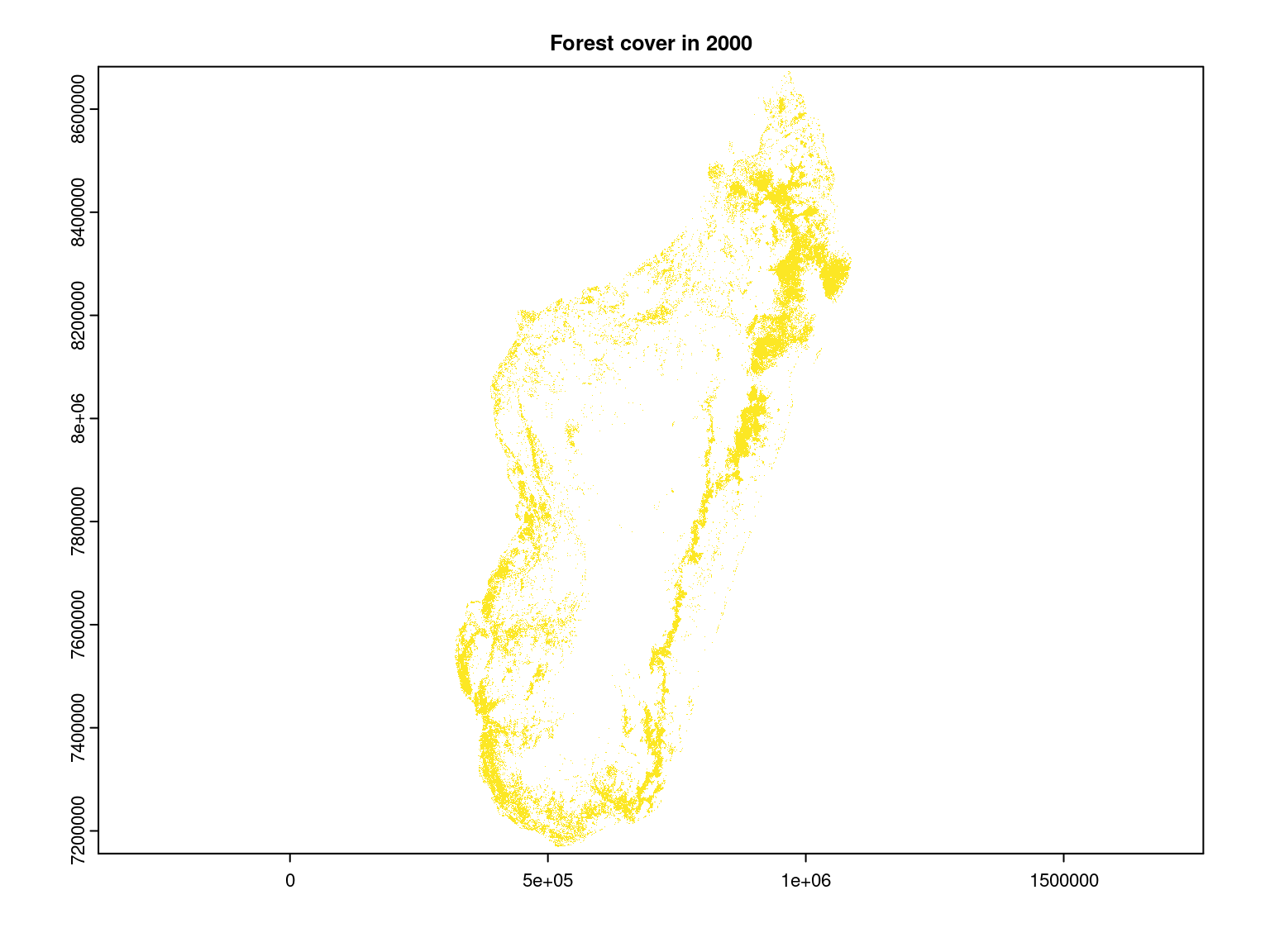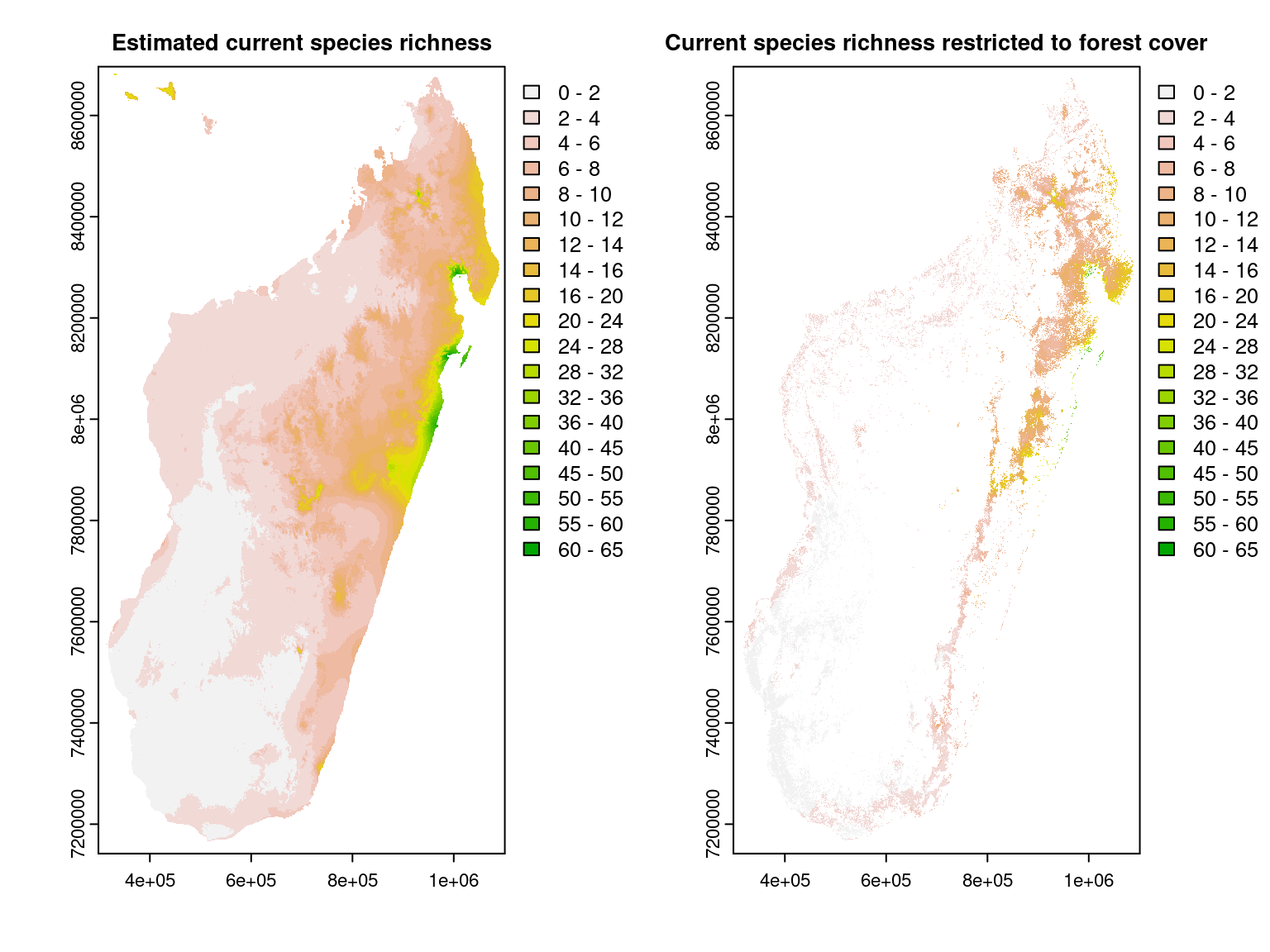We can see that the species richness, computed from interpolated occurrence probabilities, is greater in the moist forest of the north-east of the island, particularly in the Masoala peninsula as expected given the observed species richness on inventory sites. It can be explained by the fact that this area is located close to the Equator and is receiving the highest level of precipitation in Madagascar. As a consequence, this area benefits from high levels of resources (light and water) and is characterized by a low environmental stress and a long growing season . Note that the estimated species richness does not necessarily stand for a ‘true’ number of present species but represent a relative species richness between sites (one site is richer in species than another site). ### 6.3 Predictive maps of species turnover (diversity $$\beta$$) Diversity $$\beta$$ is a measure of biodiversity that compares the diversity of species between ecosystems or along environmental gradients, using the number of taxa that are unique to each ecosystem. In order to estimate this indicator, we proceed in the same way as in the article Allnutt et al. (2008) by performing a standard PCA on the interpolated probabilities of occurrence of species for each pixel of the displayed image. We use the coordinates obtained for the first three axes of the PCA that reflect the composition of the species community likely to occupy the corresponding pixel. These coordinates are scaled $$[0.255]$$ so that they can be represented by red color levels for the first axis, green for the second and blue for the third. The combination of these three color levels determines the coloring of each pixel in the displayed $$\beta$$ diversity map. Therefore, a color difference between two pixels indicates that the species present are not the same, while pixels of identical color host communities of similar species. #### 6.3.1 Current species turn-over # Sample 10000 cells of presence probabilities raster npart <- 10 theta <- terra::rast("~/Documents/projet_BioSceneMada/Report/Internship_Report/data/RST_theta_1.tif") samp_theta <- terra::spatSample(theta, size=10000, method="random", ext=terra::ext(theta), na.rm=TRUE, cells=TRUE) samp_cells <- samp_theta[,"cell"] samp_theta <- samp_theta[,-1] for(n in 2:npart){ theta <- terra::rast(paste0("~/Documents/projet_BioSceneMada/Report/Internship_Report/data/RST_theta_", n, ".tif")) samp_theta <- cbind(samp_theta, theta[samp_cells]) } params_species <- read.csv(file = "~/Documents/projet_BioSceneMada/Report/Internship_Report/data/params_species.csv") colnames(samp_theta) <- params_species$species

# Data frame to compute PCA on pixels dissimilarity
theta_df <- data.frame(samp_theta)
pca_theta <- ade4::dudi.pca(theta_df, center=TRUE, scale=TRUE, nf=3, scannf = FALSE)
str(pca_theta)

# Perform projections of PCA results on all raster's rows in parallel
# Coordinates on the 3 axes retained in the PCA
coords <- theta[[c(1,2,3)]]
terra::values(coords) <- 0
names(coords) <- colnames(pca_theta$li) for(k in 1:nrow(theta)){ cat(k, "/", nrow(theta),"\n") theta <- terra::rast("~/Documents/projet_BioSceneMada/Report/Internship_Report/data/RST_theta_1.tif") ## Make a cluster for parallel computation # detect the number of CPU cores on the current host ncores <- parallel::detectCores() clust <- makeCluster(ncores) registerDoParallel(clust) theta_k <- foreach(n=1:npart, .combine = "cbind") %dopar%{ theta <- terra::rast(paste0("~/Documents/projet_BioSceneMada/Report/Internship_Report/data/RST_theta_", n, ".tif")) theta_k <- terra::values(theta, row=k, nrows=1) return(theta_k) } stopCluster(clust) colnames(theta_k) <- params_species$species
theta_k <- data.frame(theta_k)
}
terra::writeRaster(coords,
filetype="GTiff", gdal=c("COMPRESS=LZW", "PREDICTOR=2"), overwrite=TRUE)

# Change the coordinate scale for [0.255].
## Min reduced to 0
Min <- terra::minmax(coords)["min",]
Min
coords_RGB <- coords
for (l in 1:3) {
coords_RGB[[l]] <- coords[[l]] - Min[l]
}

Min2 <- terra::minmax(coords_RGB)["min",]
Min2
## Max at 255
remove(coords)
Max <- terra::minmax(coords_RGB)["max",]
Max
for (l in 1:3) {
coords_RGB[[l]] <- (coords_RGB[[l]] / Max[l])*255
}
Max2 <- terra::minmax(coords_RGB)["max",]
Max2

# Coloration RGB
terra::writeRaster(coords_RGB,
filetype="GTiff", gdal=c("COMPRESS=LZW", "PREDICTOR=2"), overwrite=TRUE)

#### 6.3.2 Current species turn over restricted to forest cover

In the same way as above, we restrict the values obtained for diversity $$\beta$$ to the forest cover remaining in 2000.

library(glue)
# raster of Madagascar's forest cover in 2000
# current species turnover
# change resolution of species richness raster from 1000x1000m to 30x30m
cmd <- glue('gdalwarp -tr 30 30 -te {xmin(forest)} {ymin(forest)} {xmax(forest)} {ymax(forest)} -r near -co "COMPRESS=LZW" -co "PREDICTOR=2" -overwrite {in_f} {out_f}')
system(cmd)
# remove species richness values where there was no forest in 2000s
terra::writeRaster(species_turnover_deforest,
filetype="GTiff", gdal=c("COMPRESS=LZW", "PREDICTOR=2"), overwrite=TRUE)
# Representation of species turn over restricted to forest cover in 2000's
par(mfrow=c(1,2))
terra::plotRGB(species_turnover_current, stretch="hist",
mar=c(1,1,1,1),
main="Estimated current species turn over")
terra::plotRGB(species_turnover_deforest, stretch="hist",
mar=c(1,1,1,1),
main="Current species turn over on forest cover")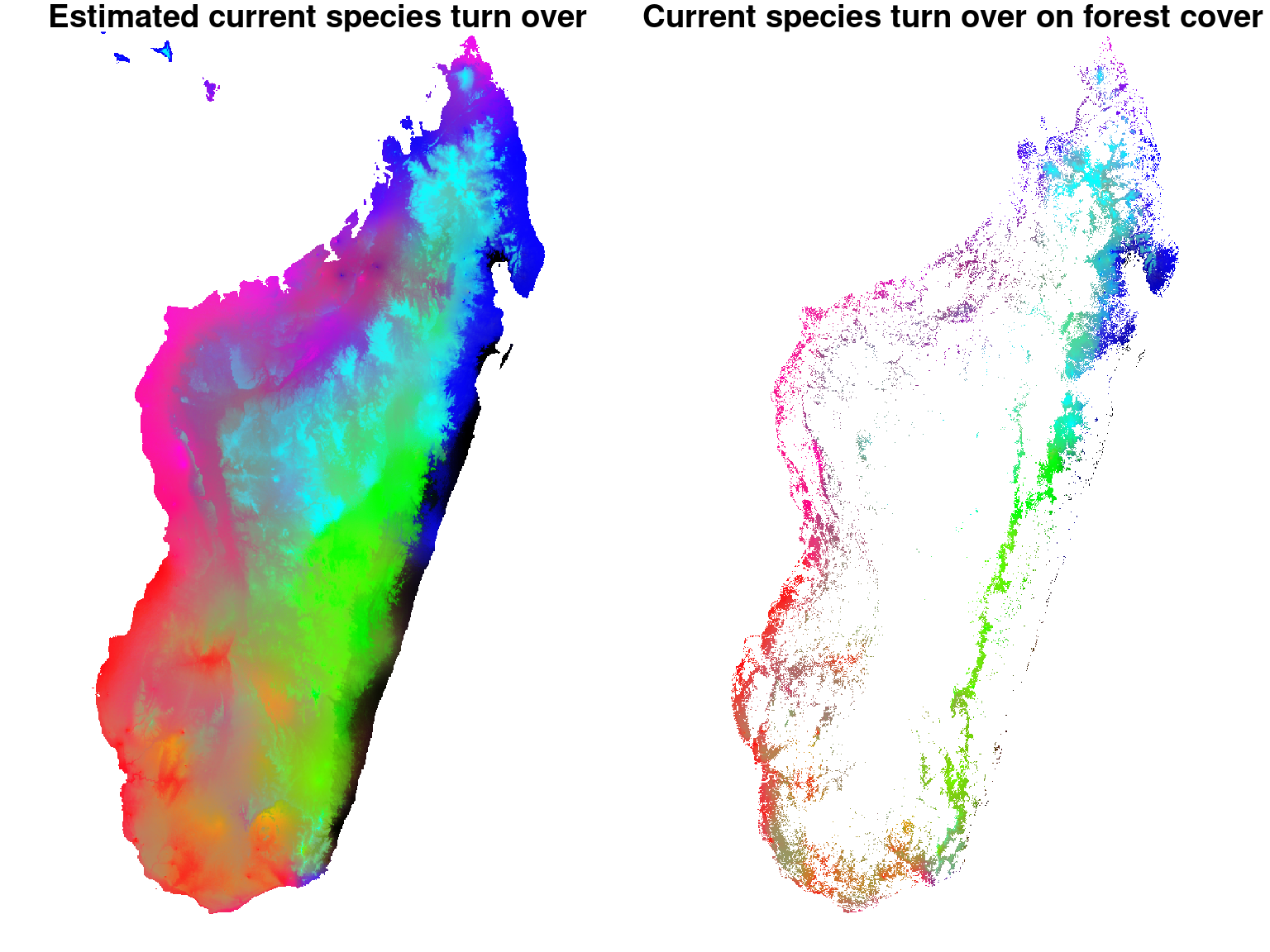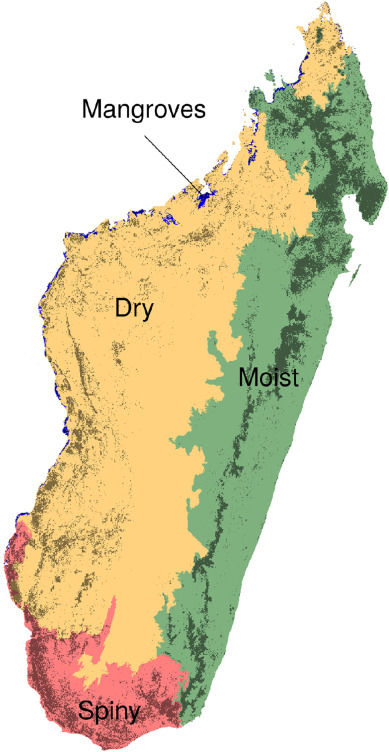Figure 6.1: Forest types in Madagascar .

We can see that the estimated map of $$\beta$$-diversity agrees with the map of Madagascar’s forest types from the article Vieilledent et al. (2018).

Indeed, communities of species represented by shades of blue and green correspond to the eastern moist forest, communities represented by shades of red match the western dry forest and communities in magenta correspond to the southern spiny forest.

Moreover, the $$\beta$$-diversity map we obtain allows to identify substantially different species communities within these three forest types. For example, we can clearly identify three different tree communities (in blue, green, and black) within the eastern moist forest.

Albert, J.H. & Siddhartha, C. (1993) Bayesian analysis of binary and polychotomous response data. Journal of the American Statistical Association, 88, 669–679.
Allnutt, T.F., Ferrier, S., Manion, G., Powell, G.V.N., Ricketts, T.H., Fisher, B.L., Harper, G.J., Irwin, M.E., Kremen, C., Labat, J.-N., Lees, D.C., Pearce, T.A. & Rakotondrainibe, F. (2008) A method for quantifying biodiversity loss and its application to a 50-year record of deforestation across Madagascar. Conservation Letters, 1, 173–181.
Mitášová, H. & Hofierka, J. (1993) Interpolation by regularized spline with tension: II. Application to terrain modeling and surface geometry analysis. Mathematical Geology, 25, 657–669.
Scherrer, D., Mod, H.K. & Guisan, A. (2020) How to evaluate community predictions without thresholding? Methods in Ecology and Evolution, 11, 51–63.
Vieilledent, G., Cornu, C., Cuní Sanchez, A., Leong Pock-Tsy, J.-M. & Danthu, P. (2013) Vulnerability of baobab species to climate change and effectiveness of the protected area network in Madagascar: Towards new conservation priorities. Biological Conservation, 166, 11–22.
Vieilledent, G., Grinand, C., Rakotomalala, F.A., Ranaivosoa, R., Rakotoarijaona, J.-R., Allnutt, T.F. & Achard, F. (2018) Combining global tree cover loss data with historical national forest cover maps to look at six decades of deforestation and forest fragmentation in Madagascar. Biological Conservation, 222, 189–197.
Warton, D.I., Blanchet, F.G., O’Hara, R.B., Ovaskainen, O., Taskinen, S., Walker, S.C. & Hui, F.K.C. (2015) So many variables: Joint modeling in community ecology. Trends in Ecology & Evolution, 30, 766–779.